# Madhya Pradesh Board Question Paper for Class 10th Maths 2019 In PDF

## MP Board (MPBSE) Question Paper 2019 Class 10th Mathematics For English Medium with Solutions – Free Download

MP board question paper 2019 class 10th maths with solutions are presented on this page in a downloadable pdf format and also in the text, so that the students can easily prepare for their MPBSE 10th class exams. MP Board Maths Question Paper 2019 for Class 10th is also attached here for reference. In addition, students will be able to access all the MP board previous year maths question papers. These papers, uploaded by BYJU’S, will act as a guideline for the students to schedule their preparation and also as to how they should solve the questions in the MP board examination.

### MPBSE Class 10th Maths Question Paper With Solutions 2019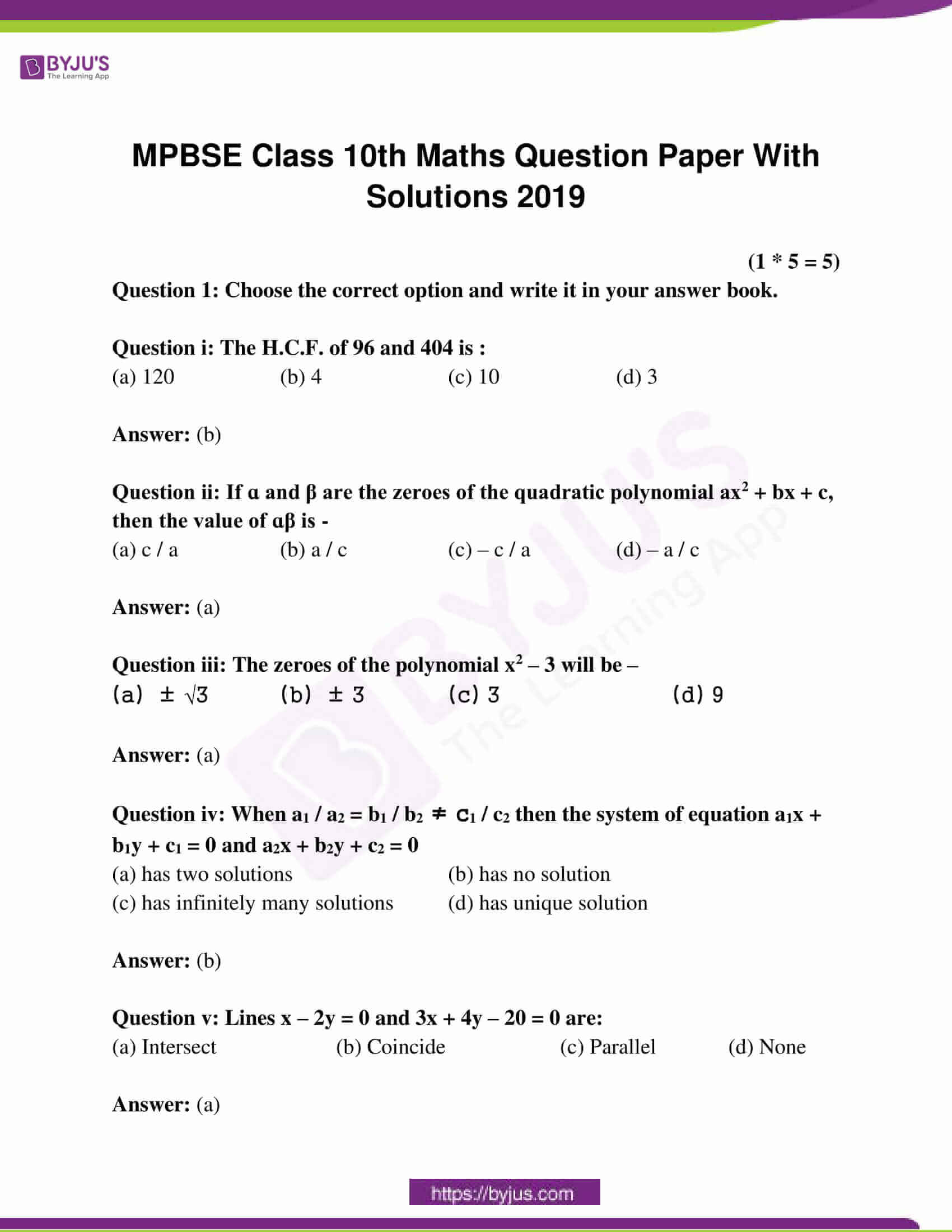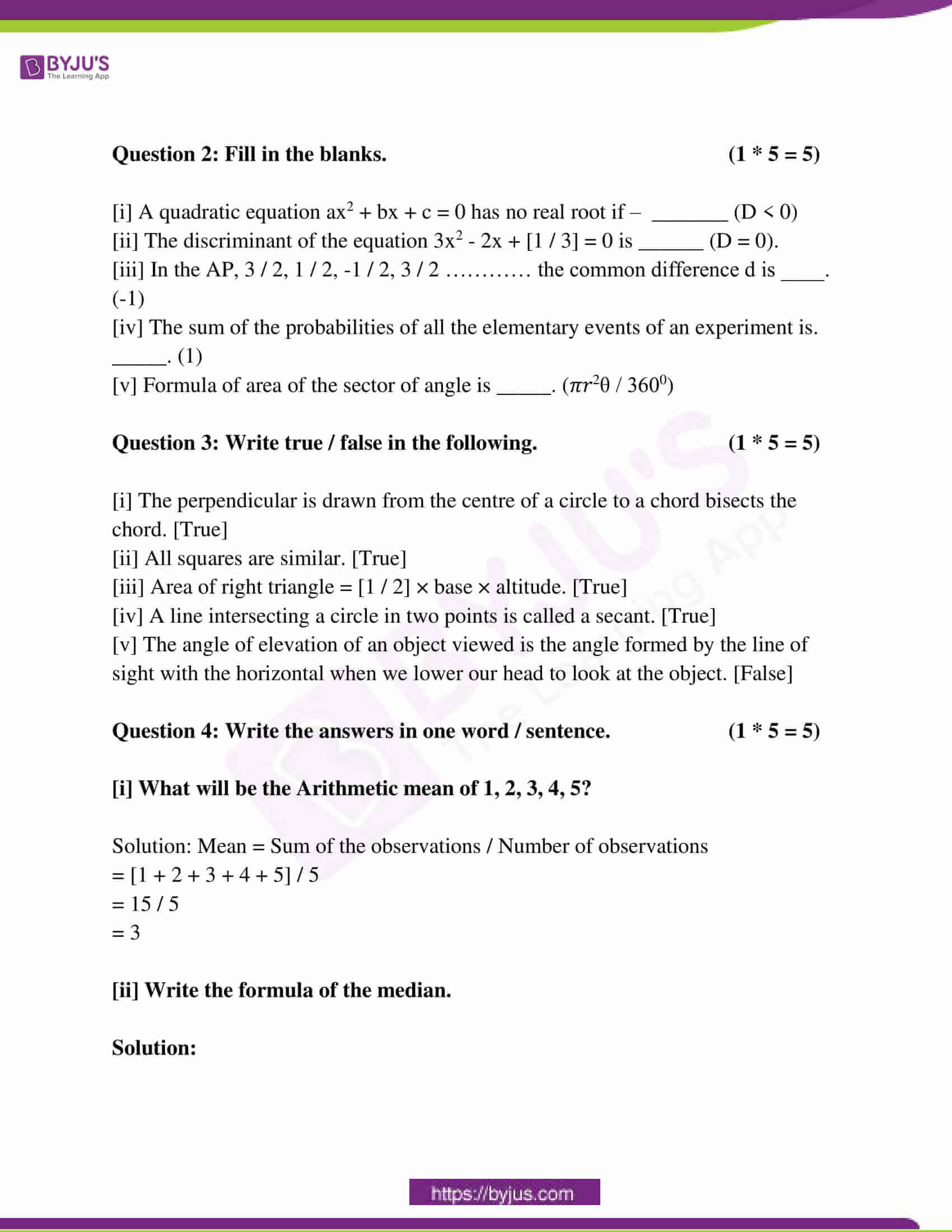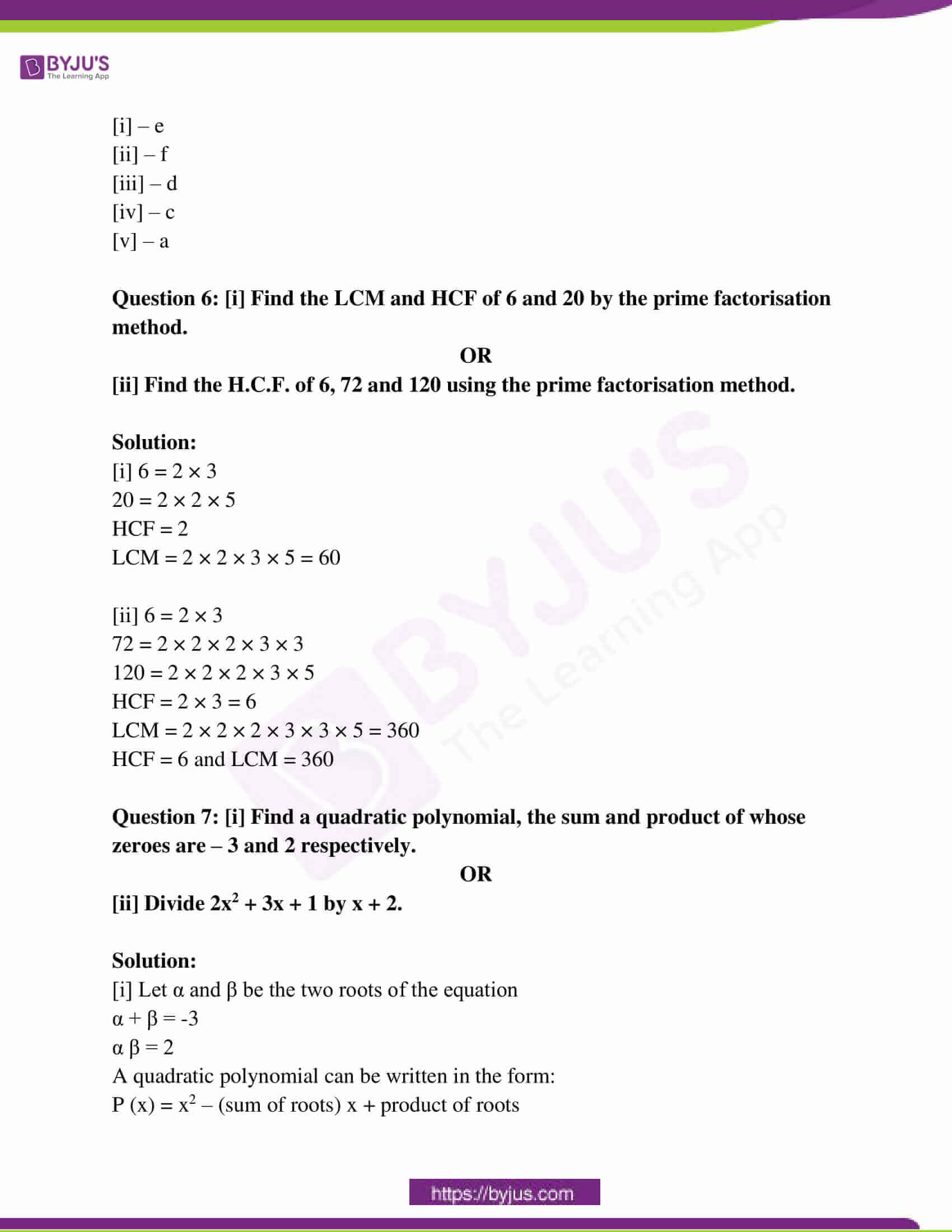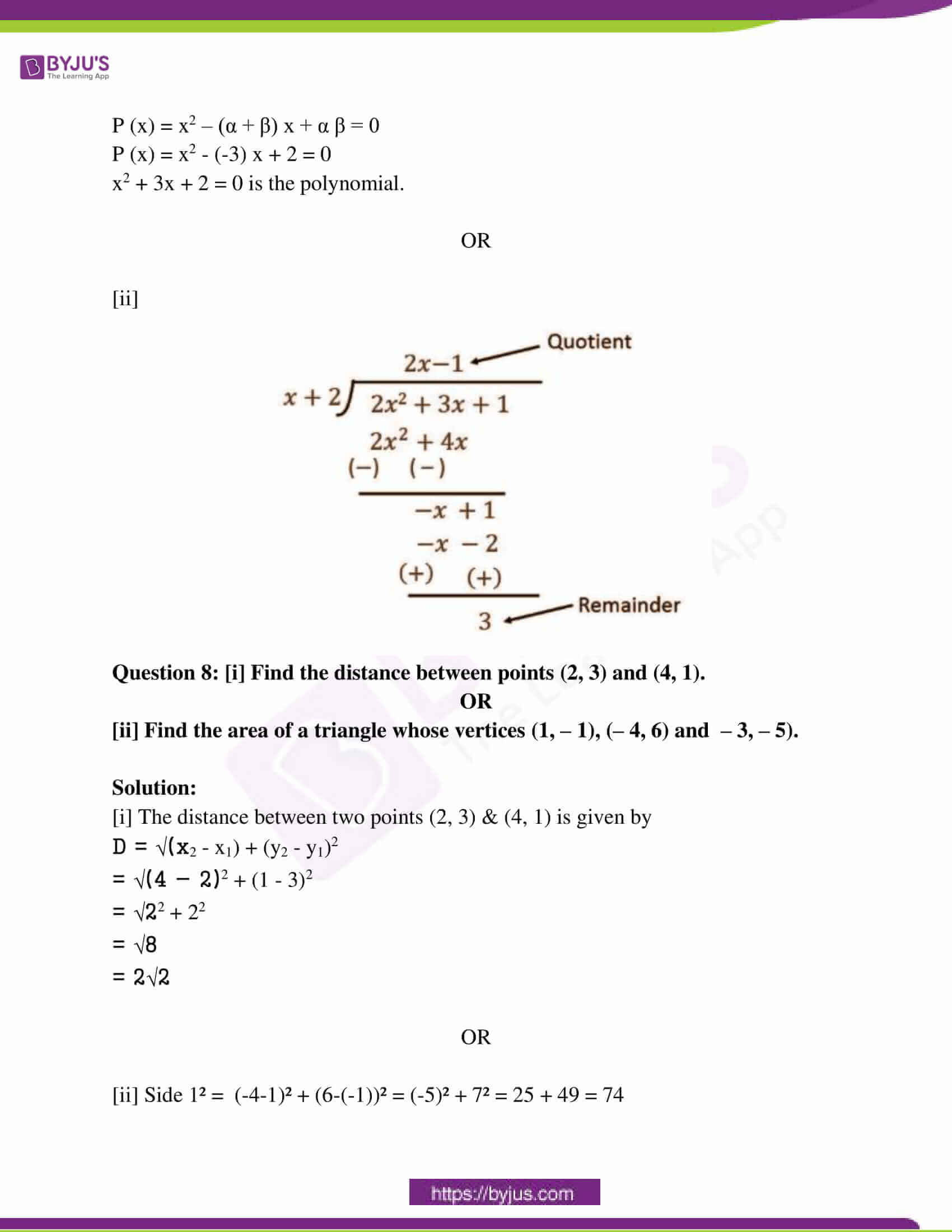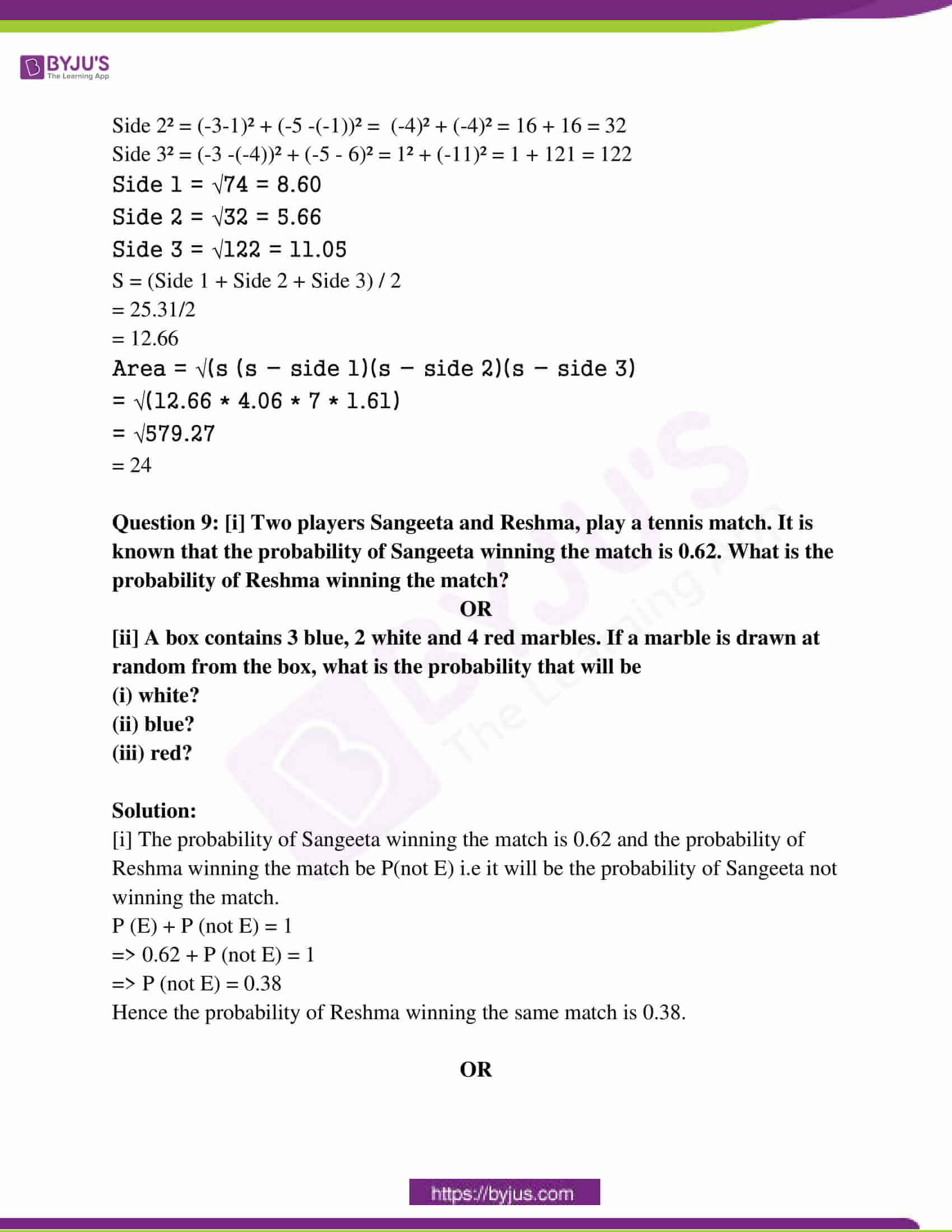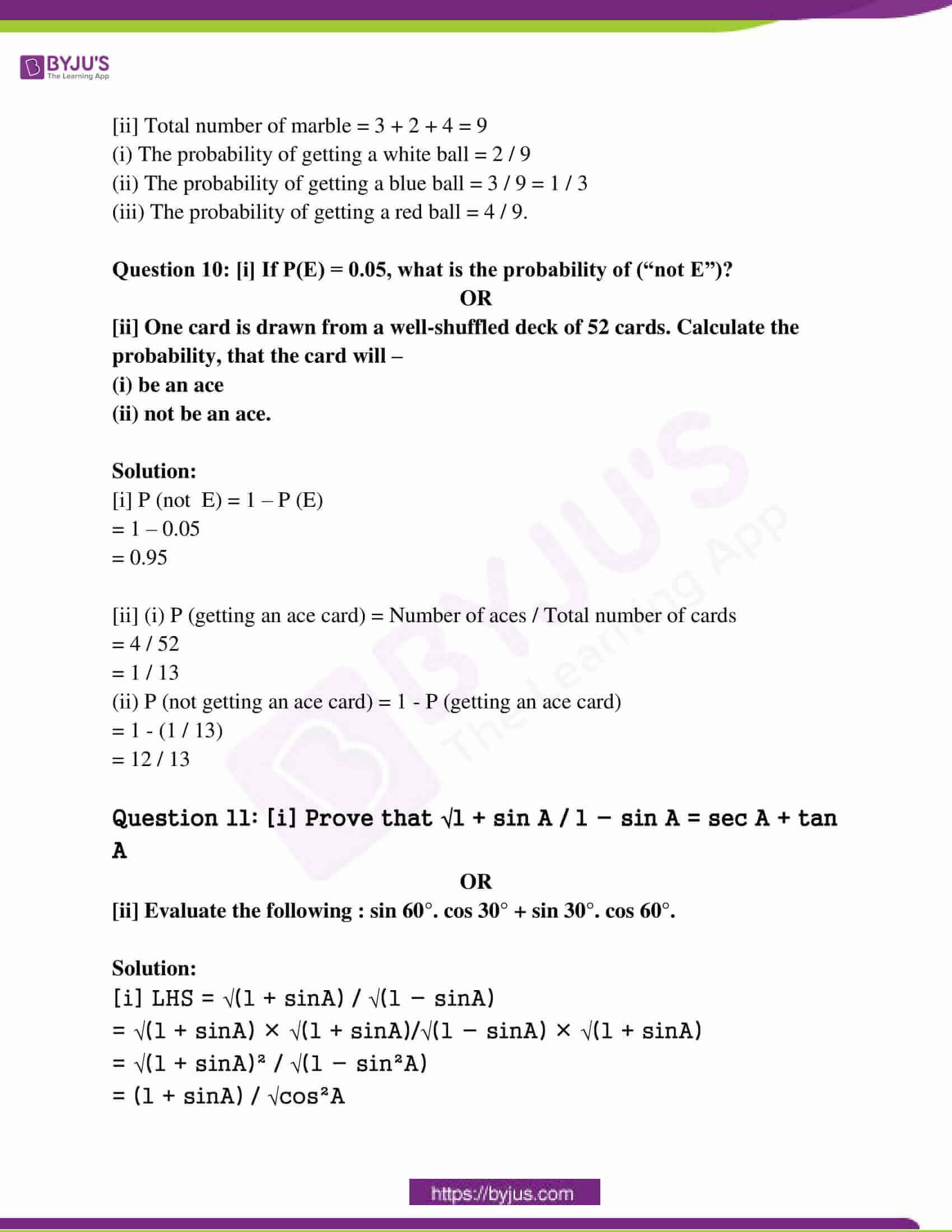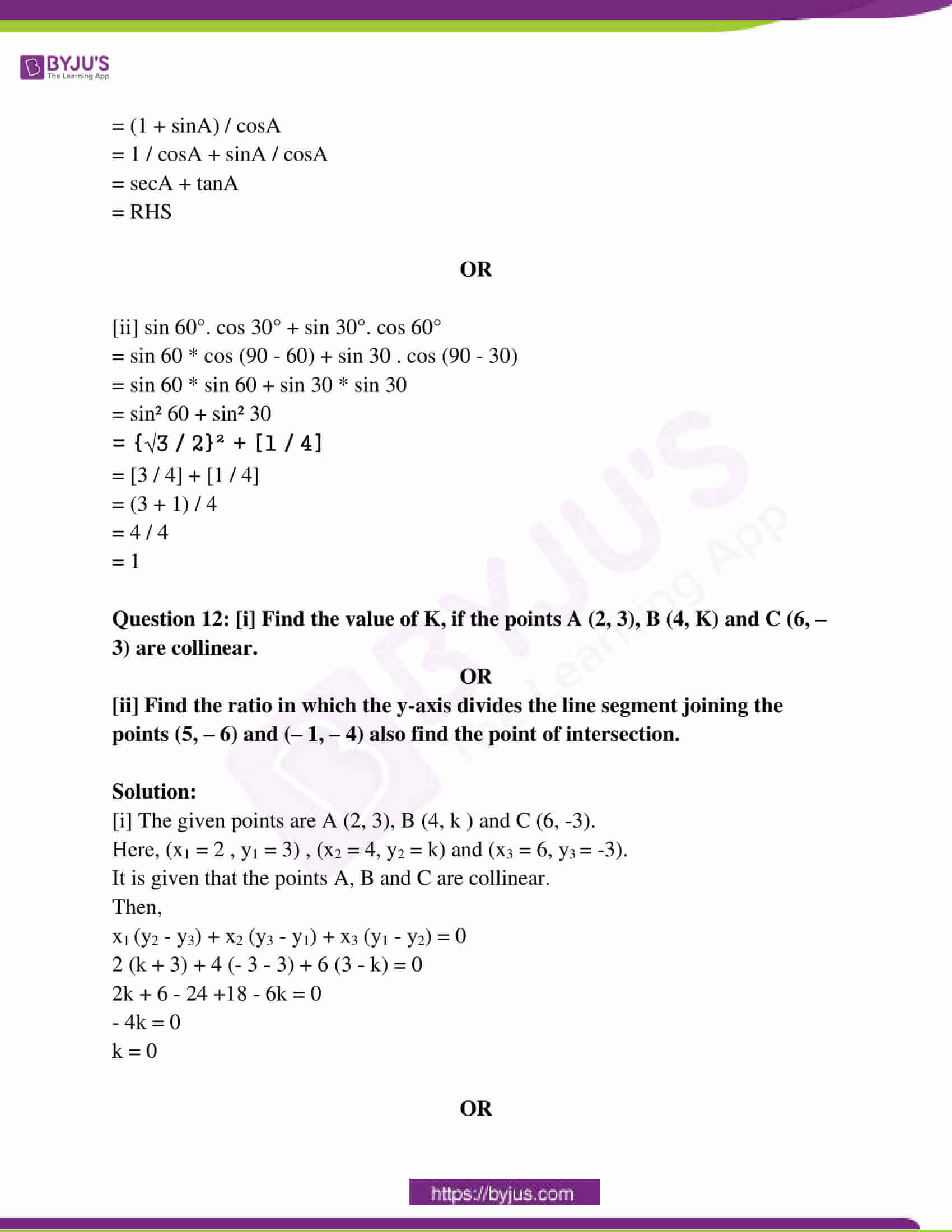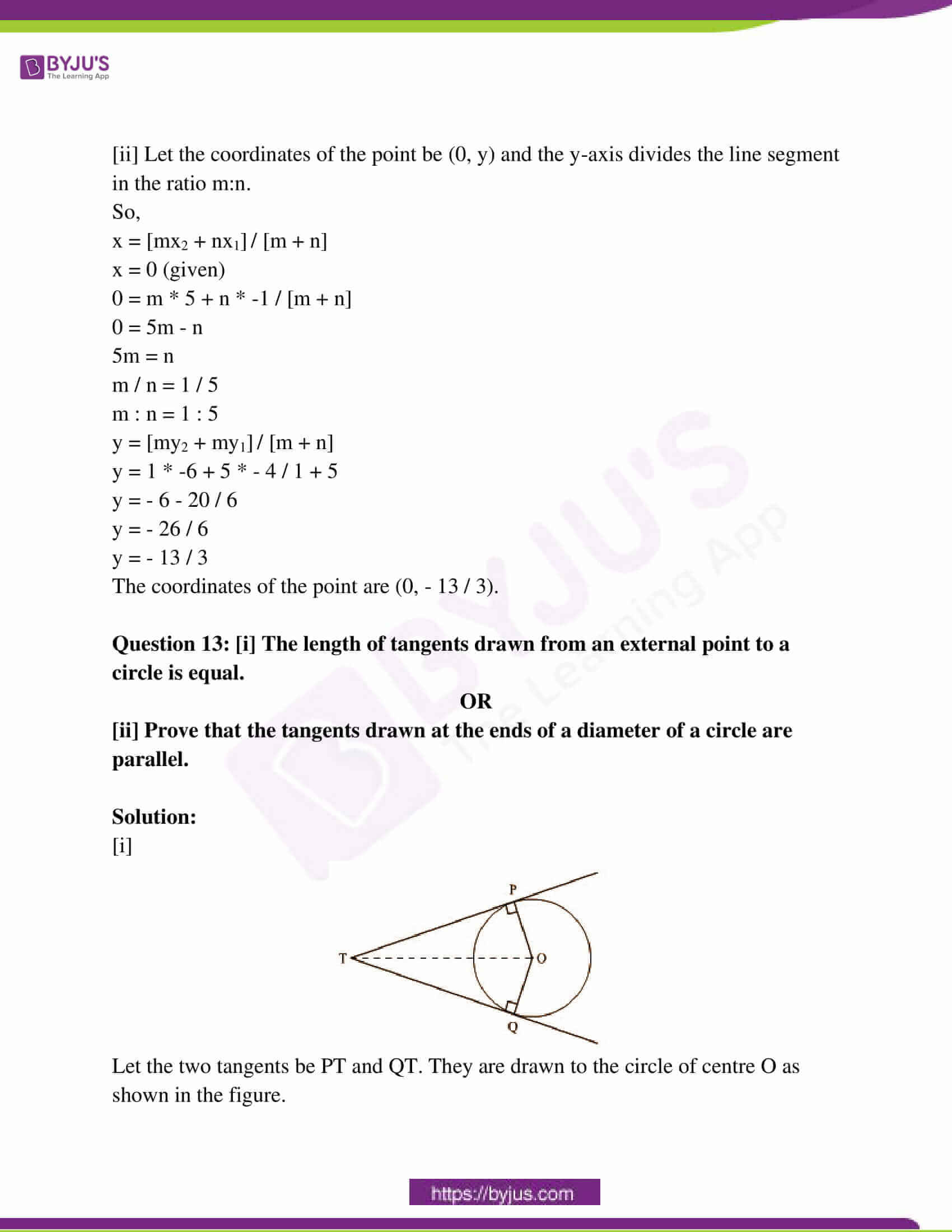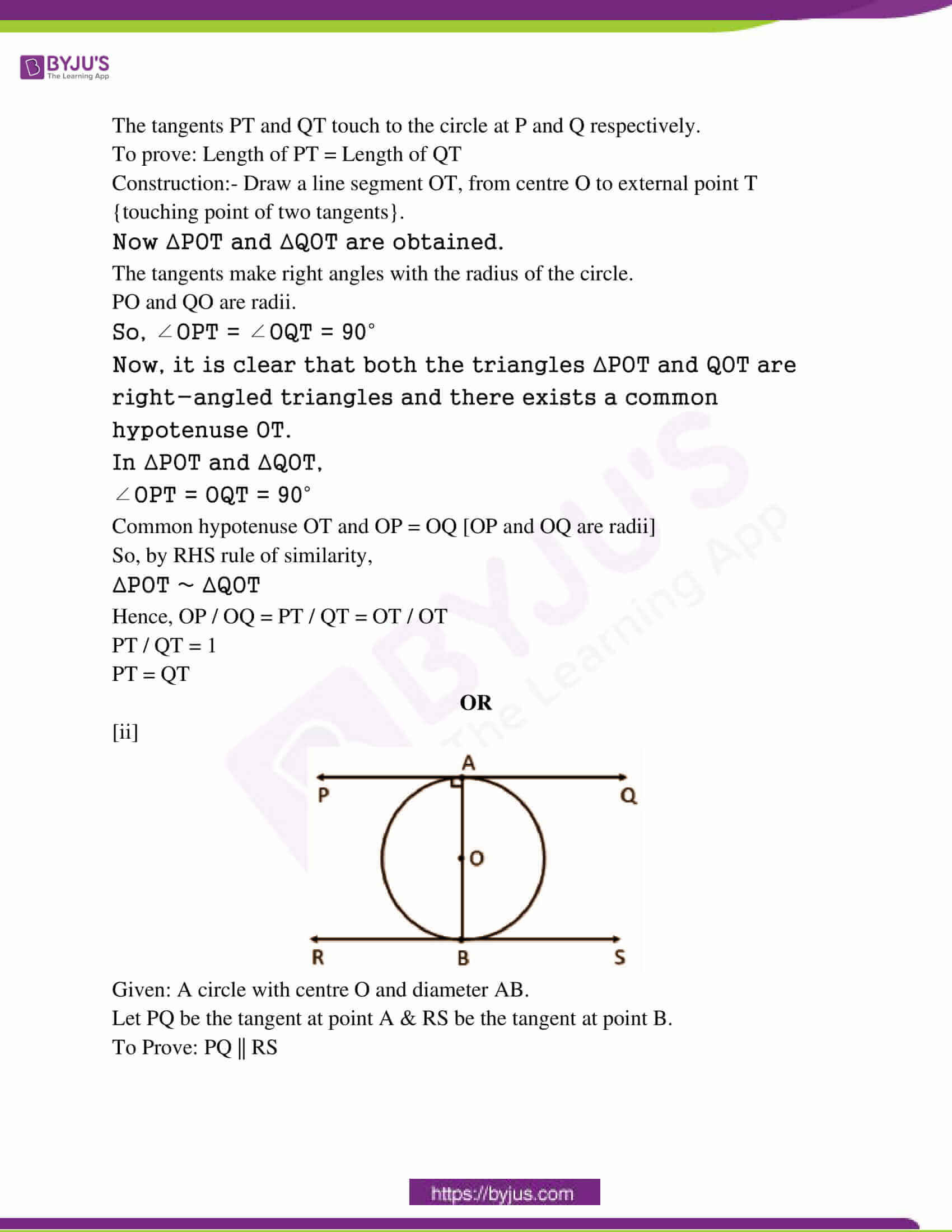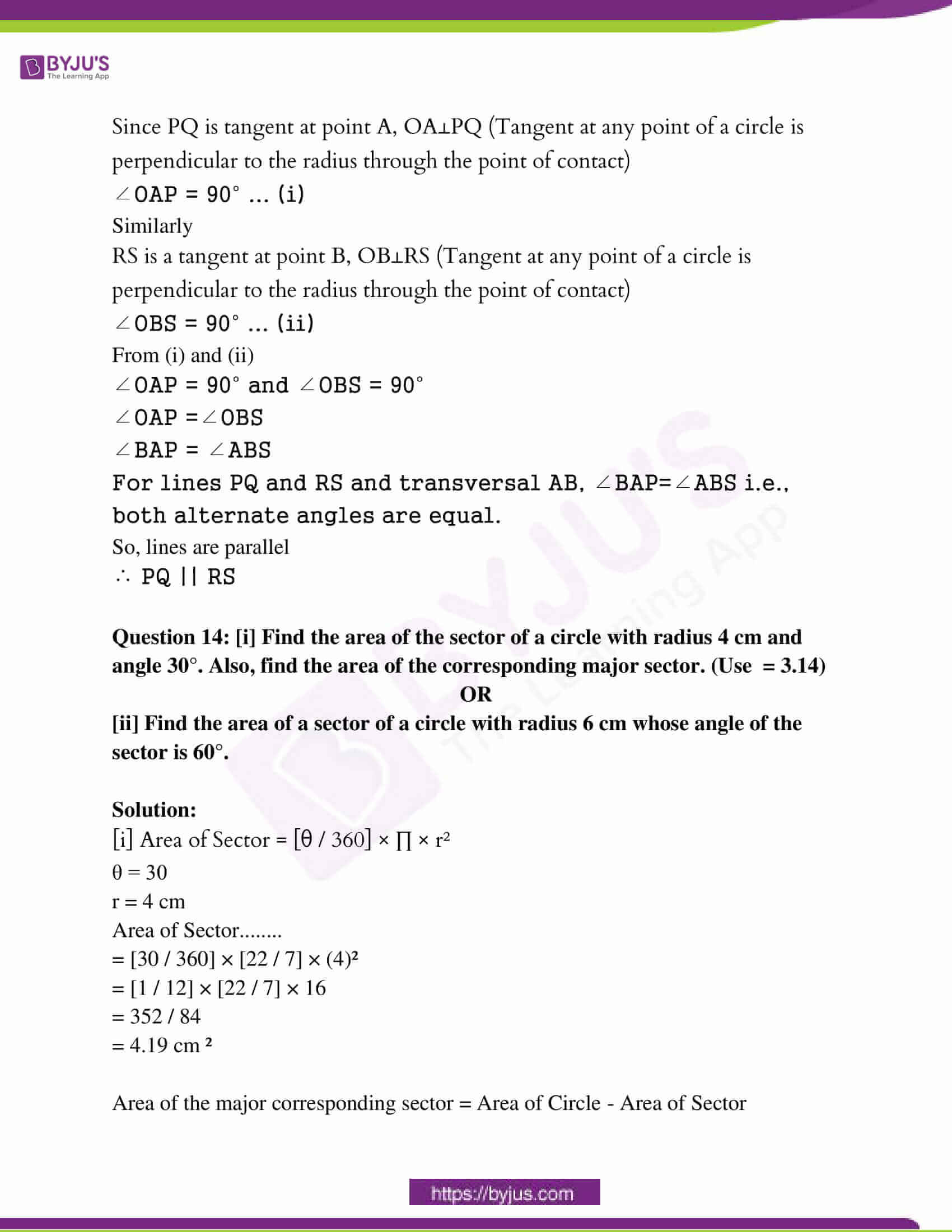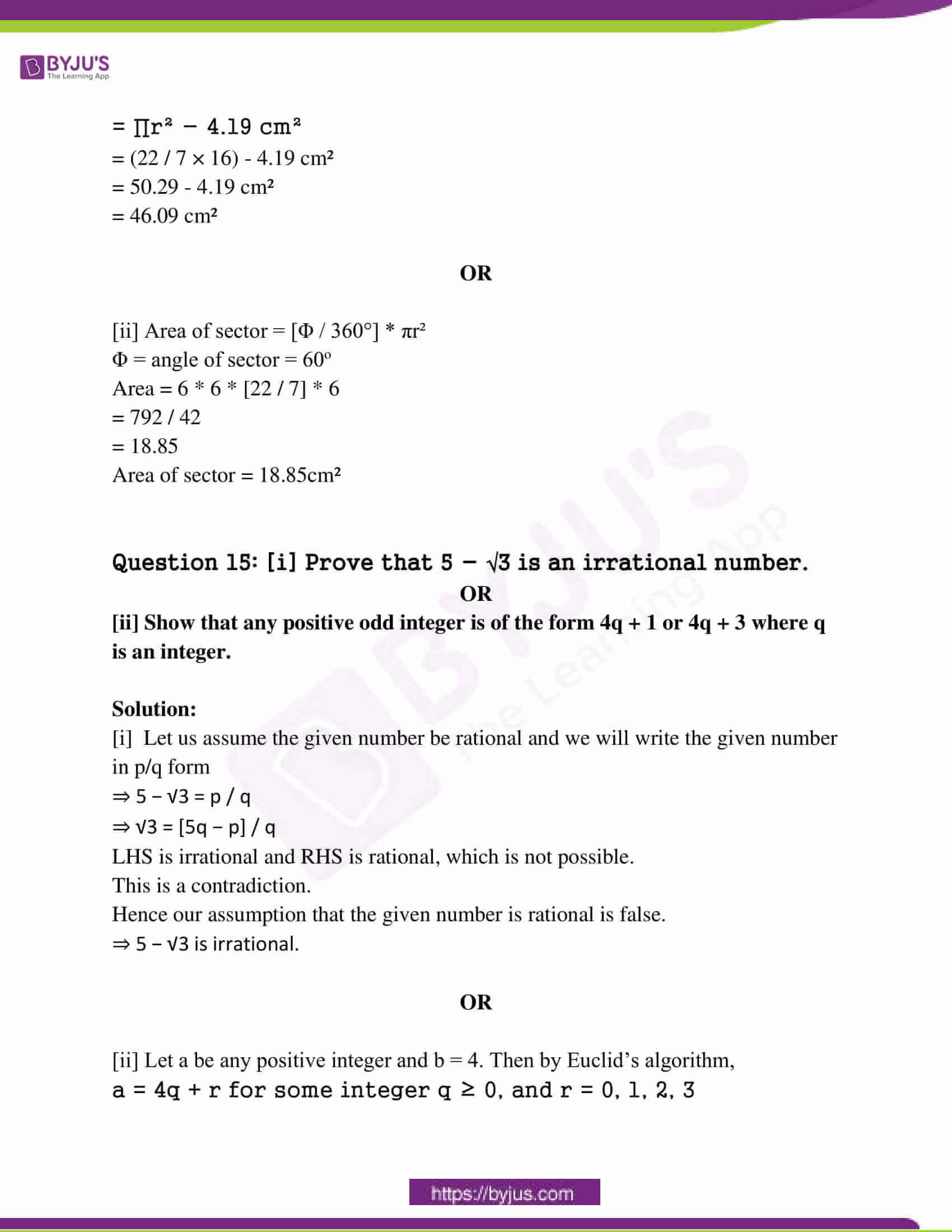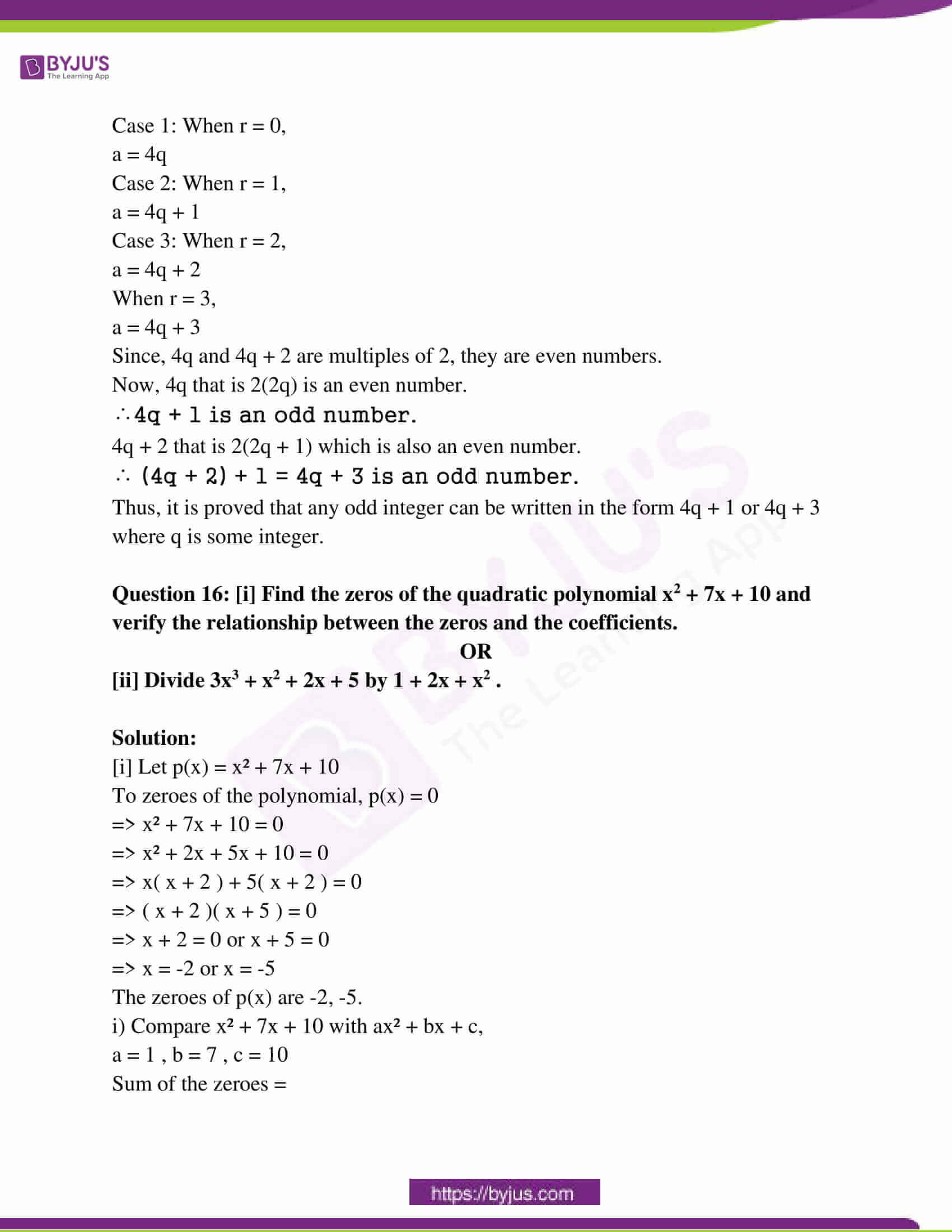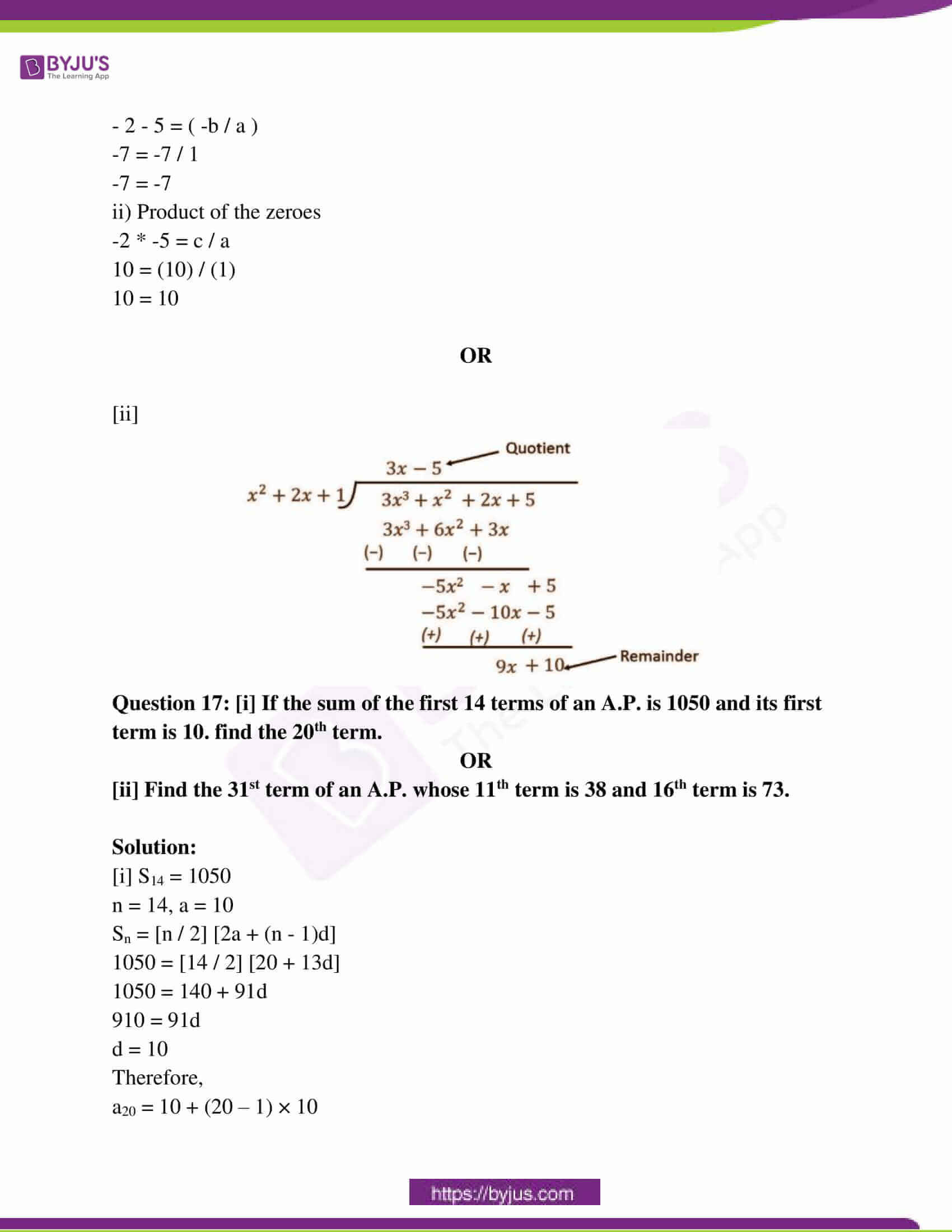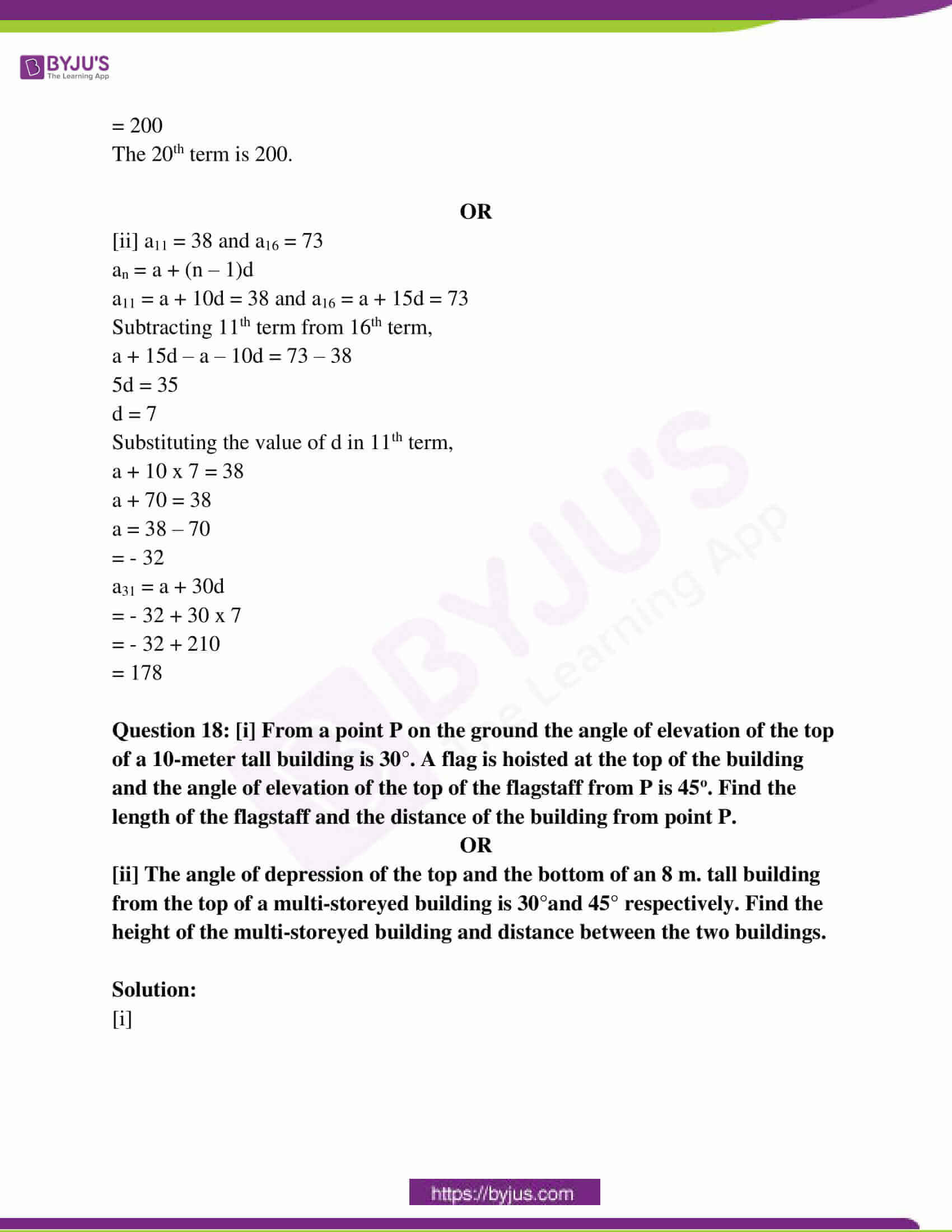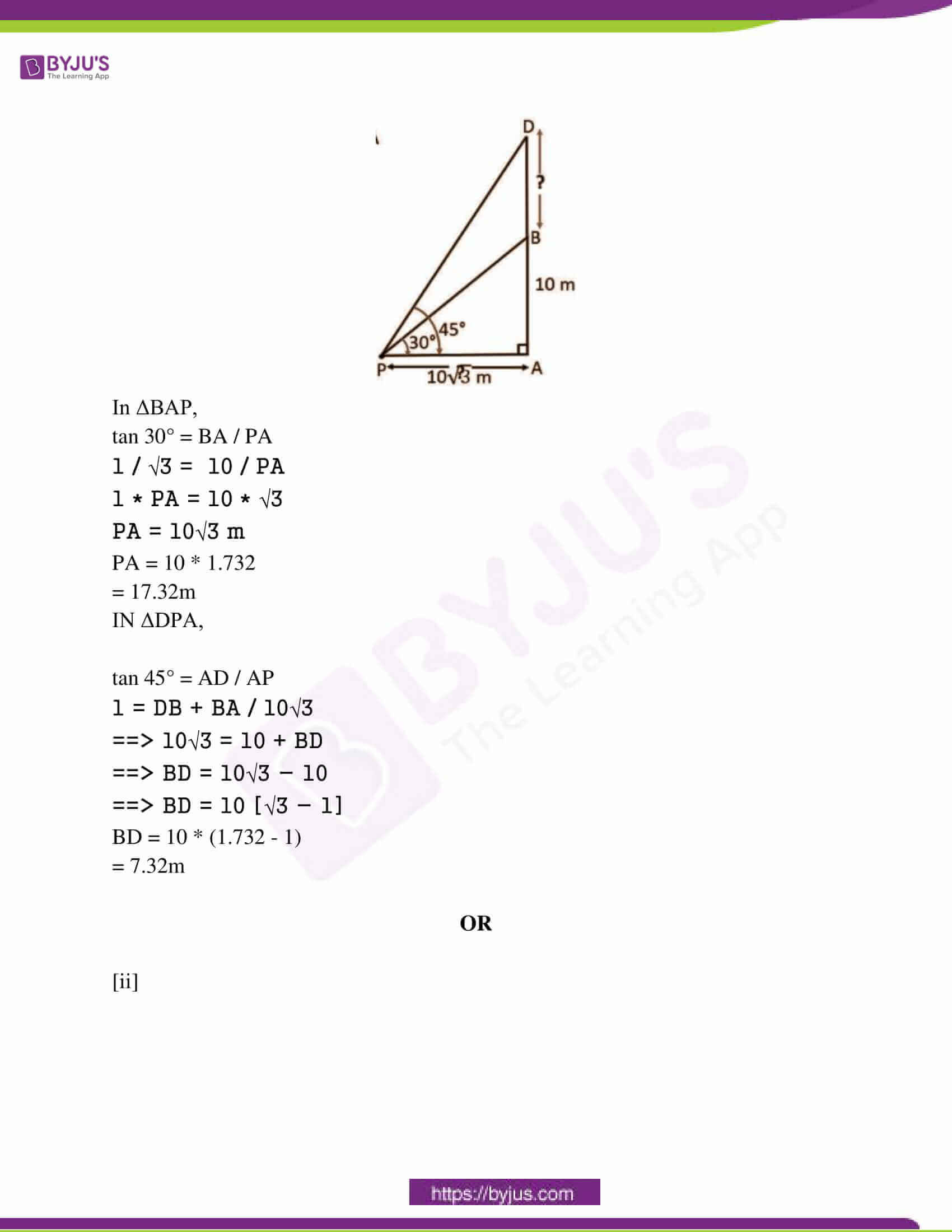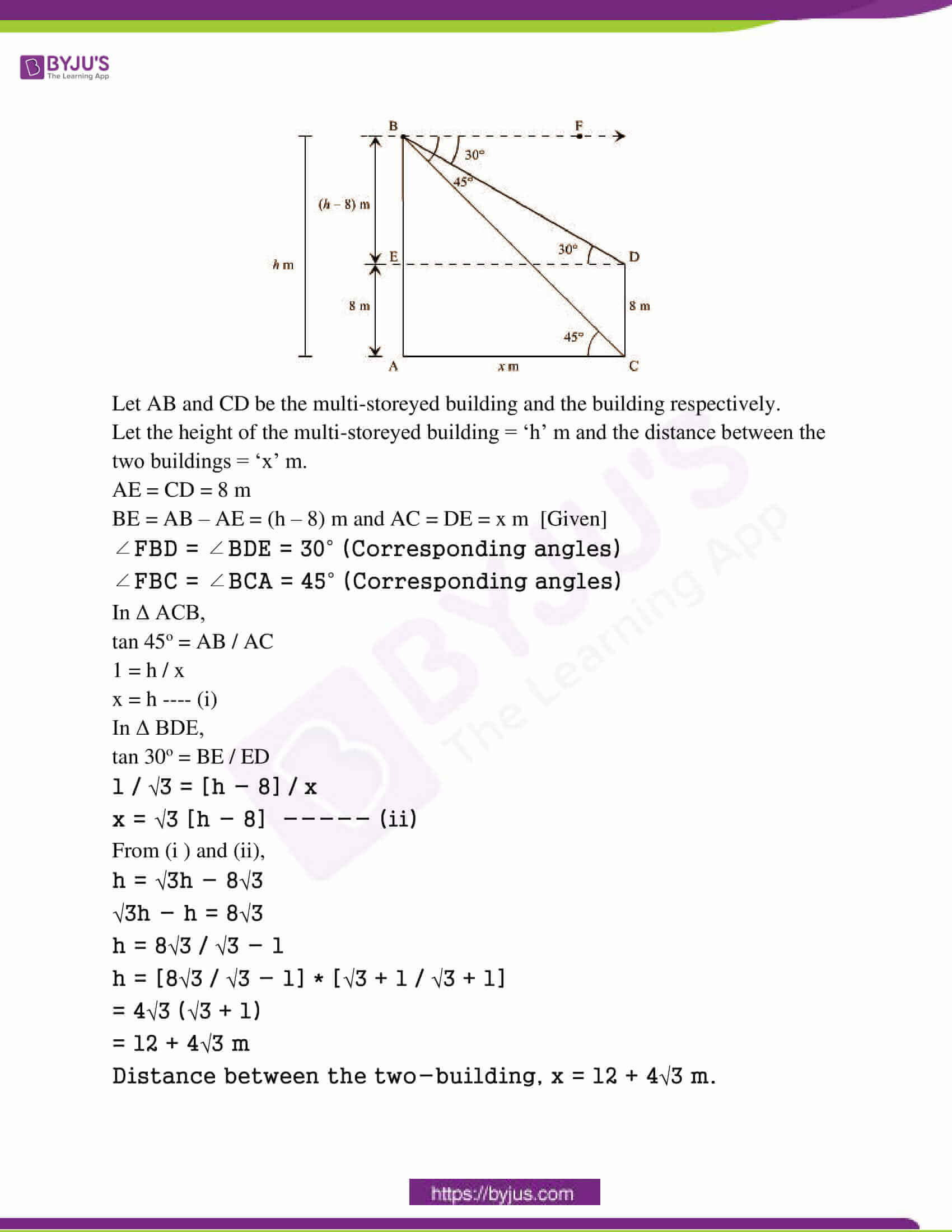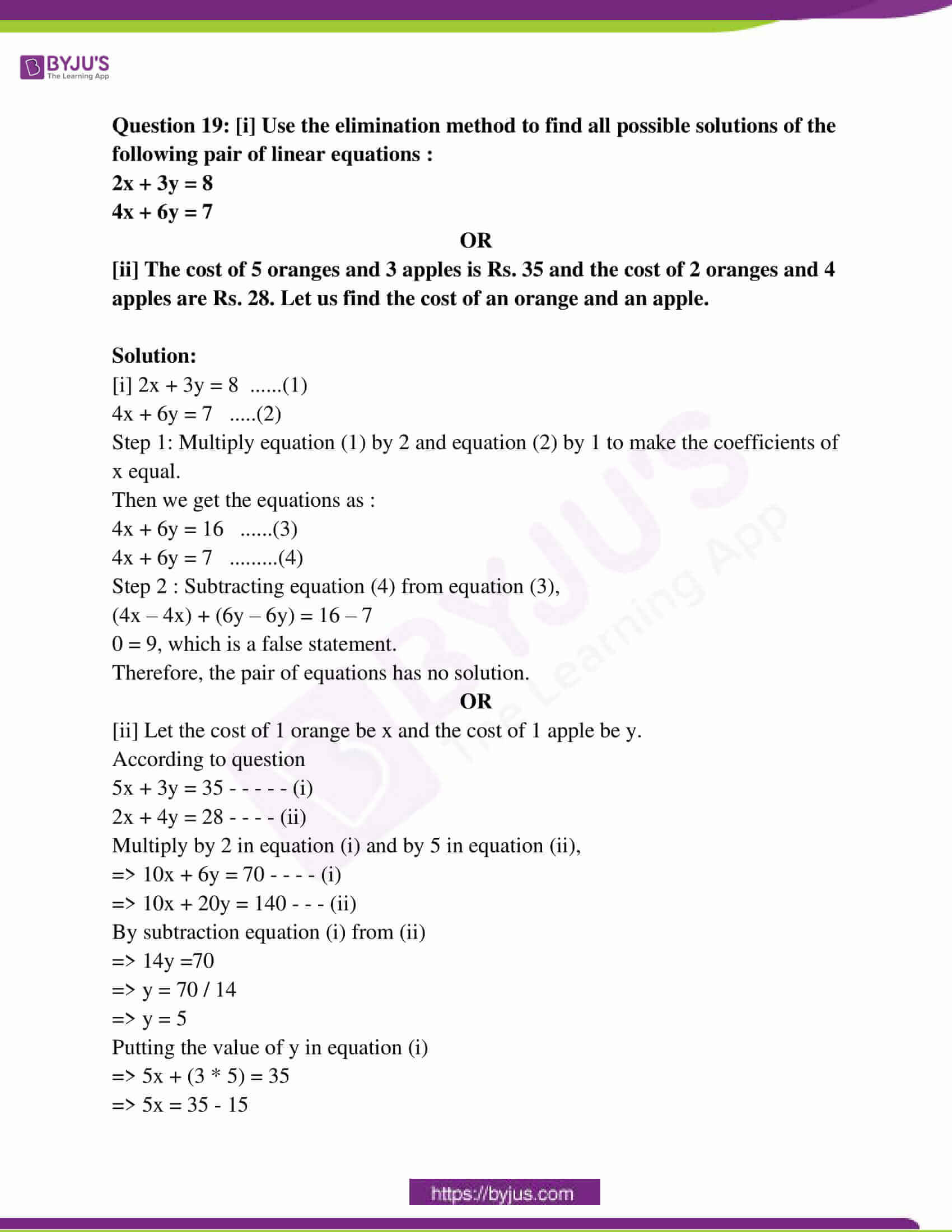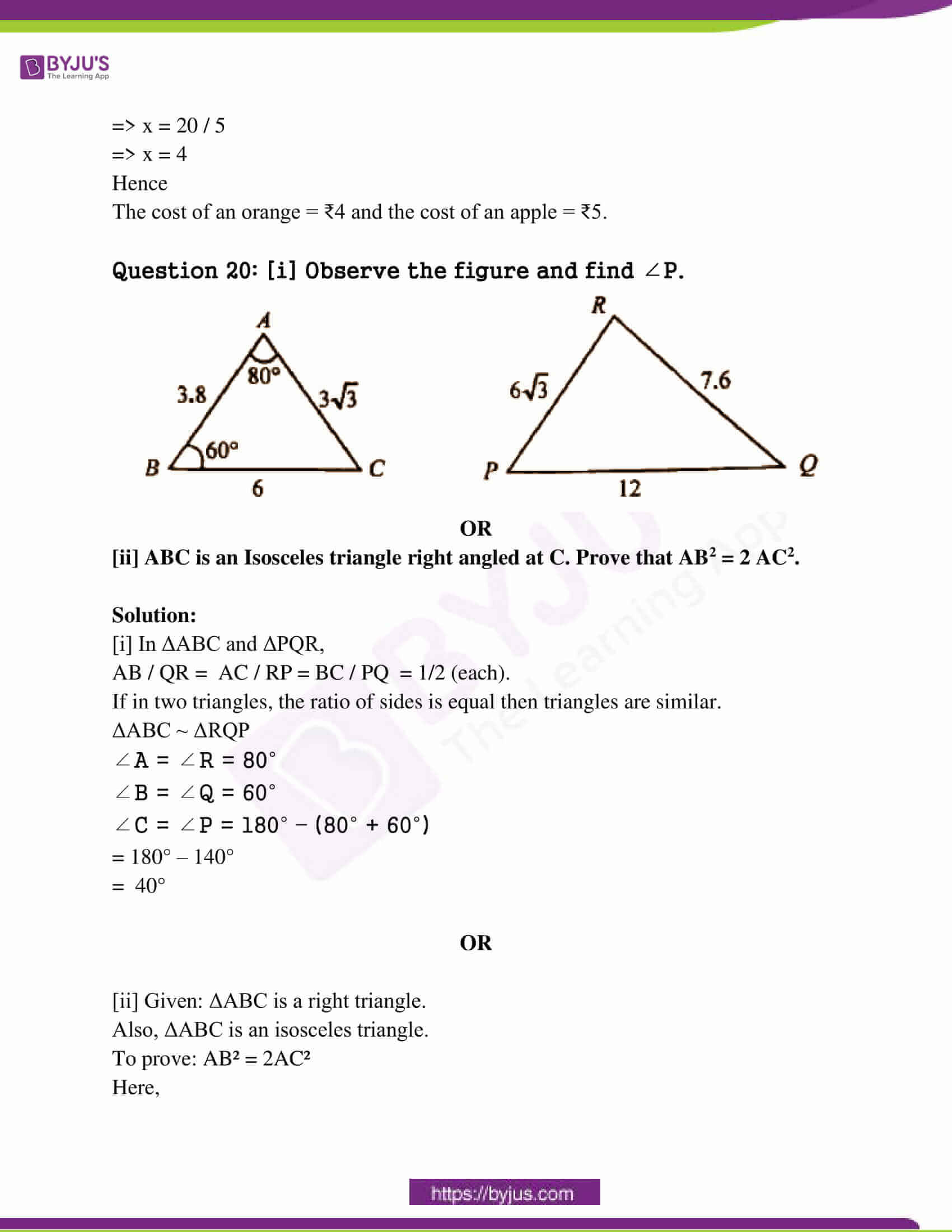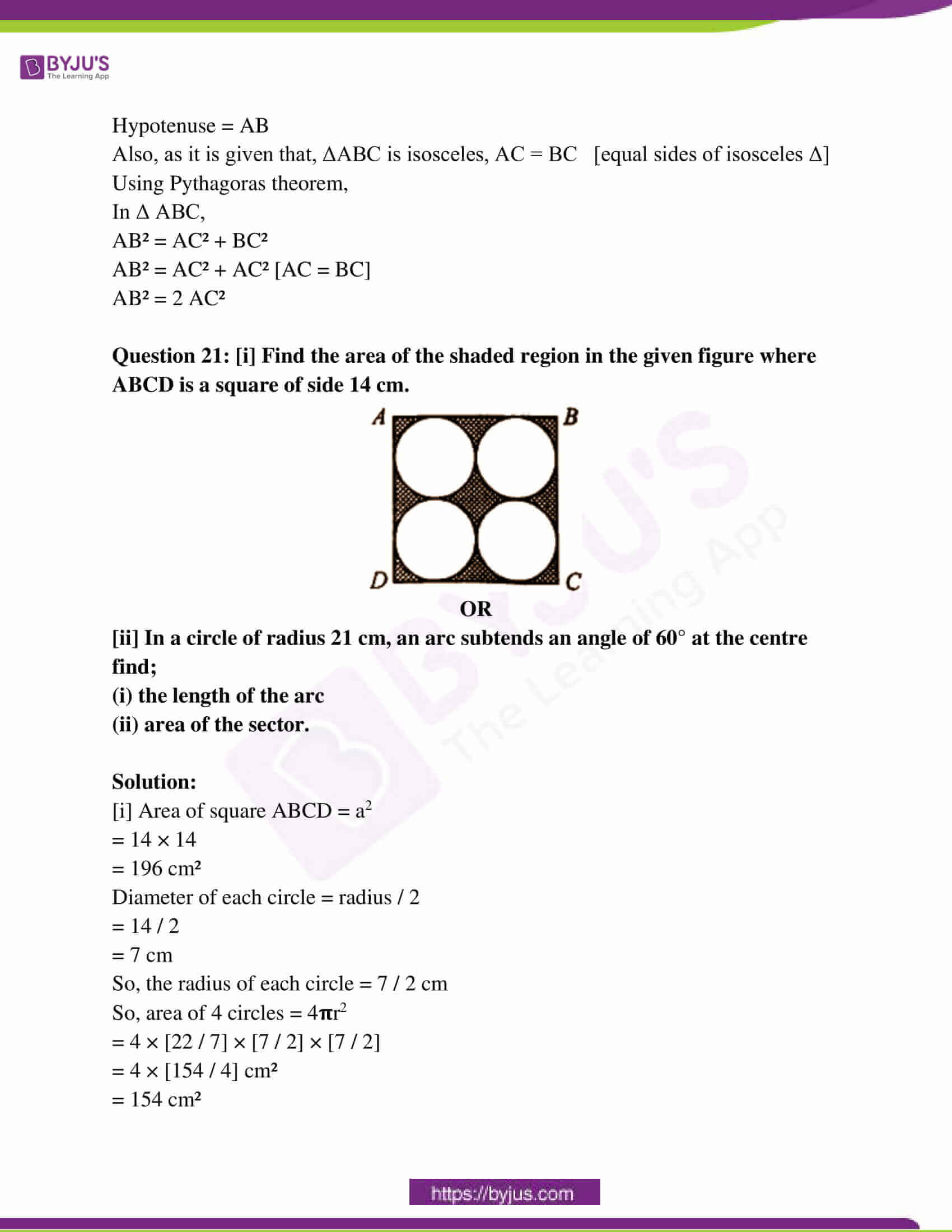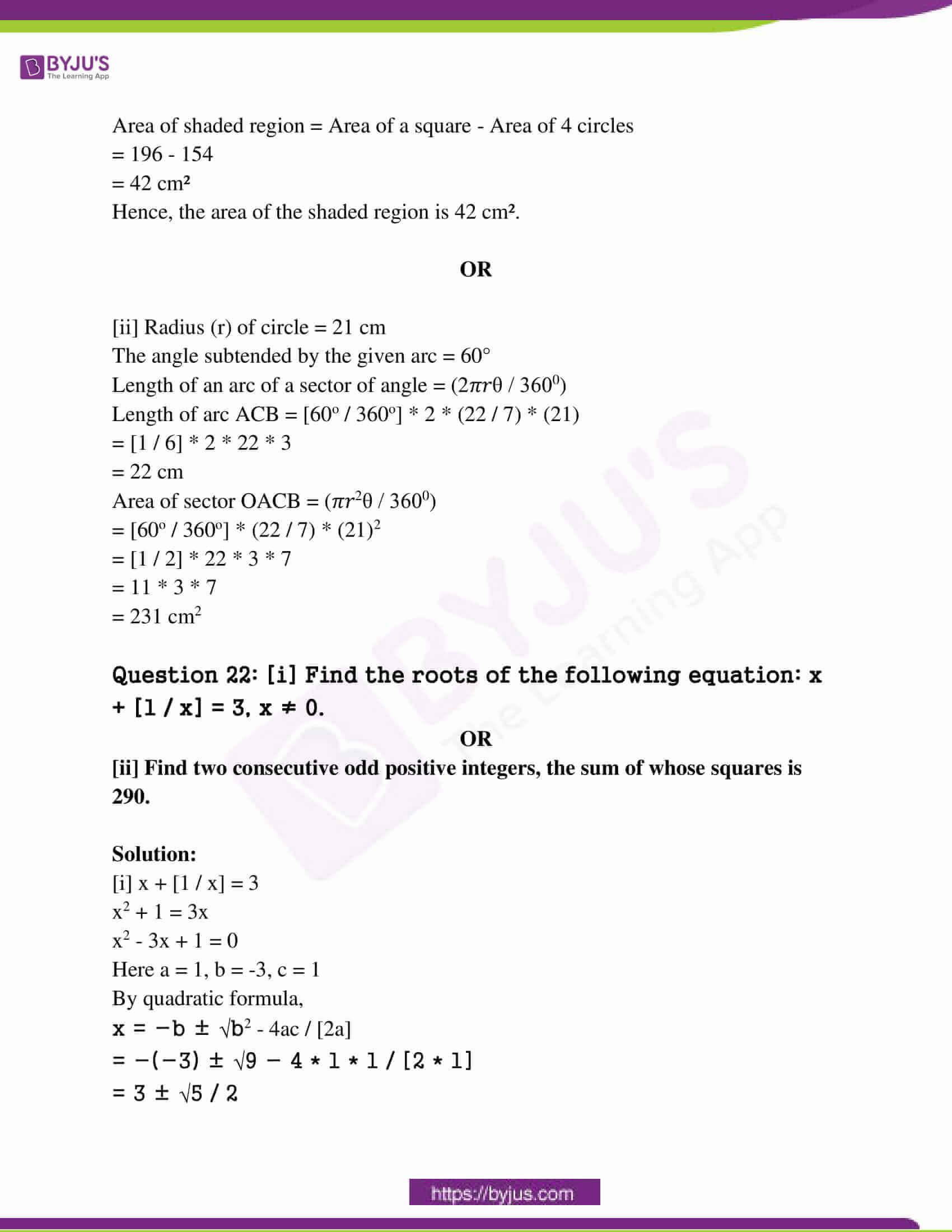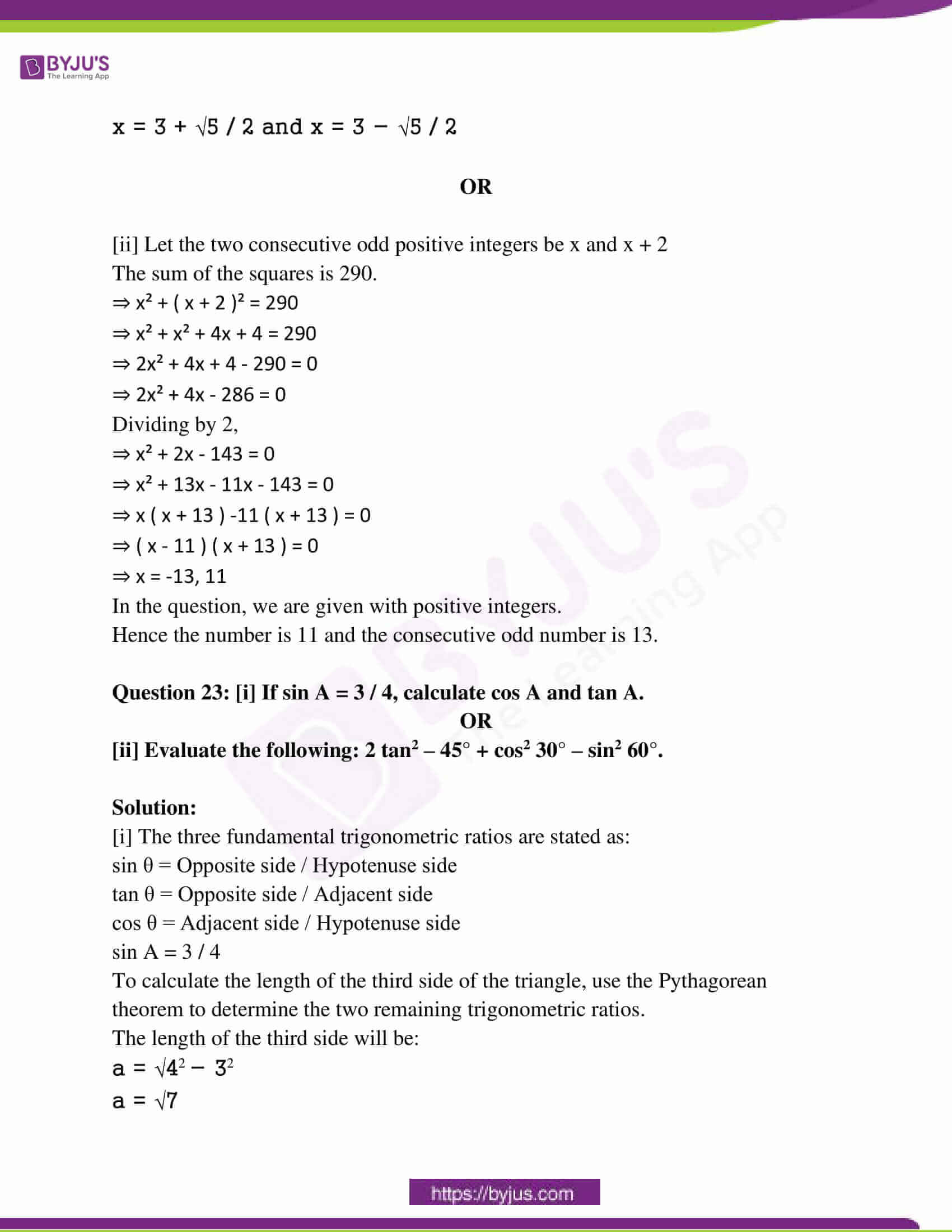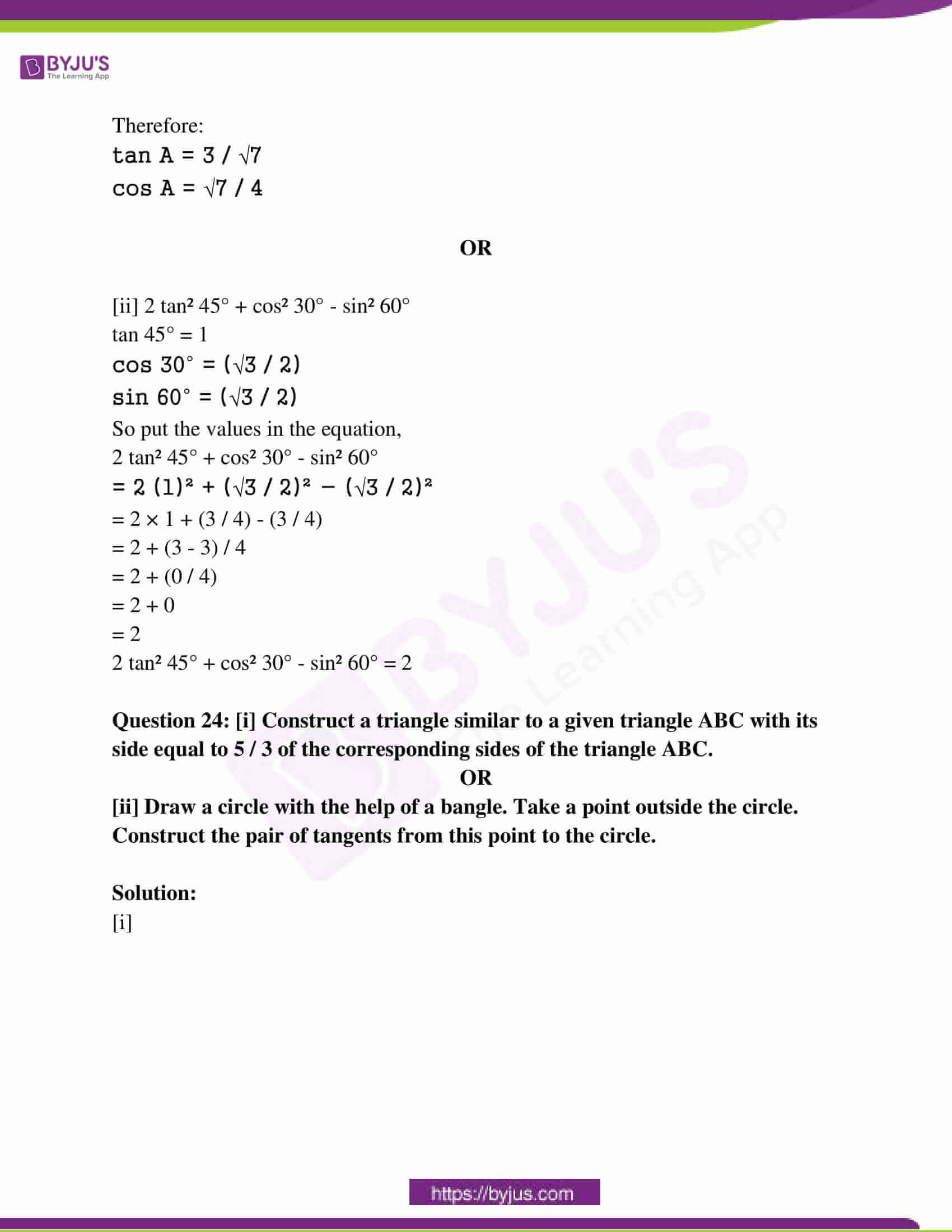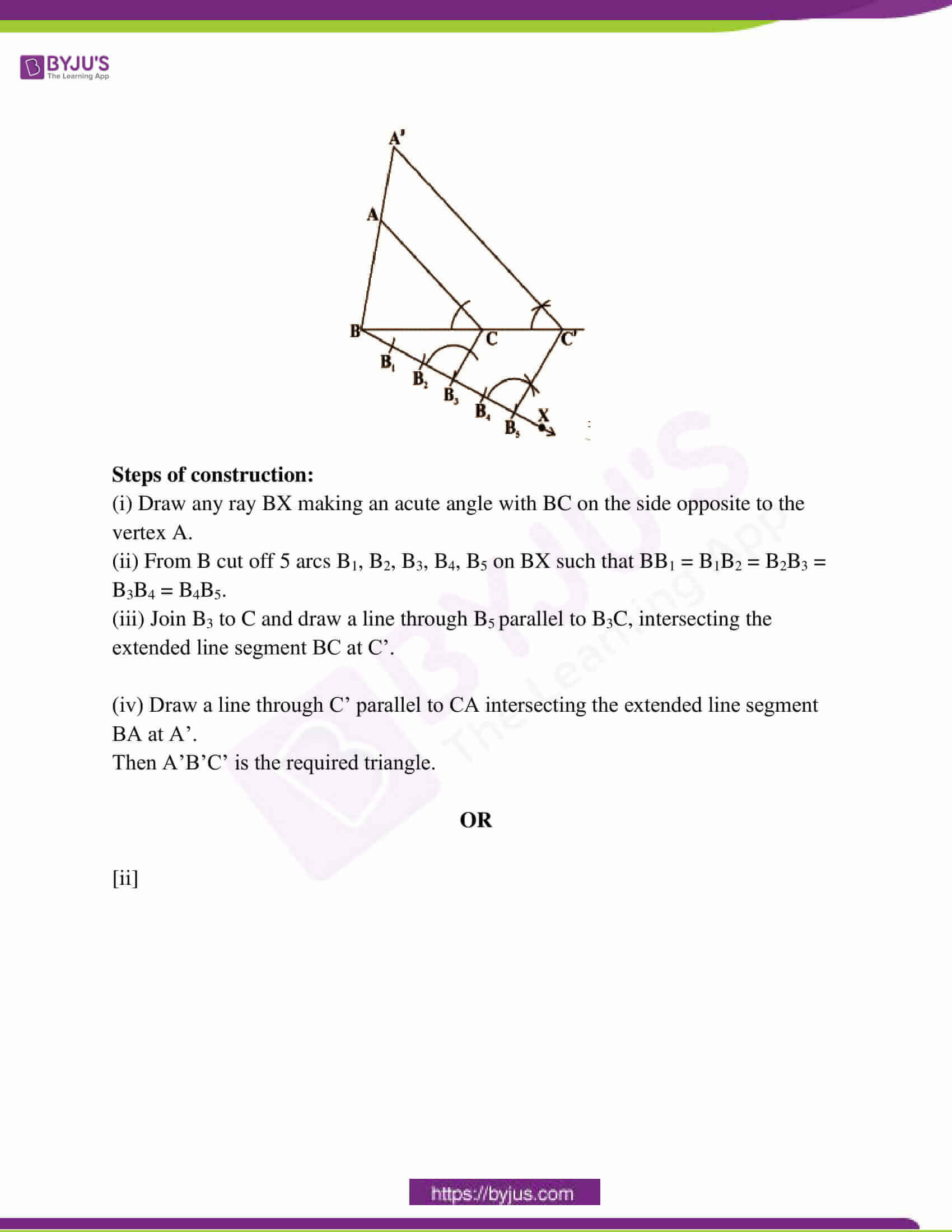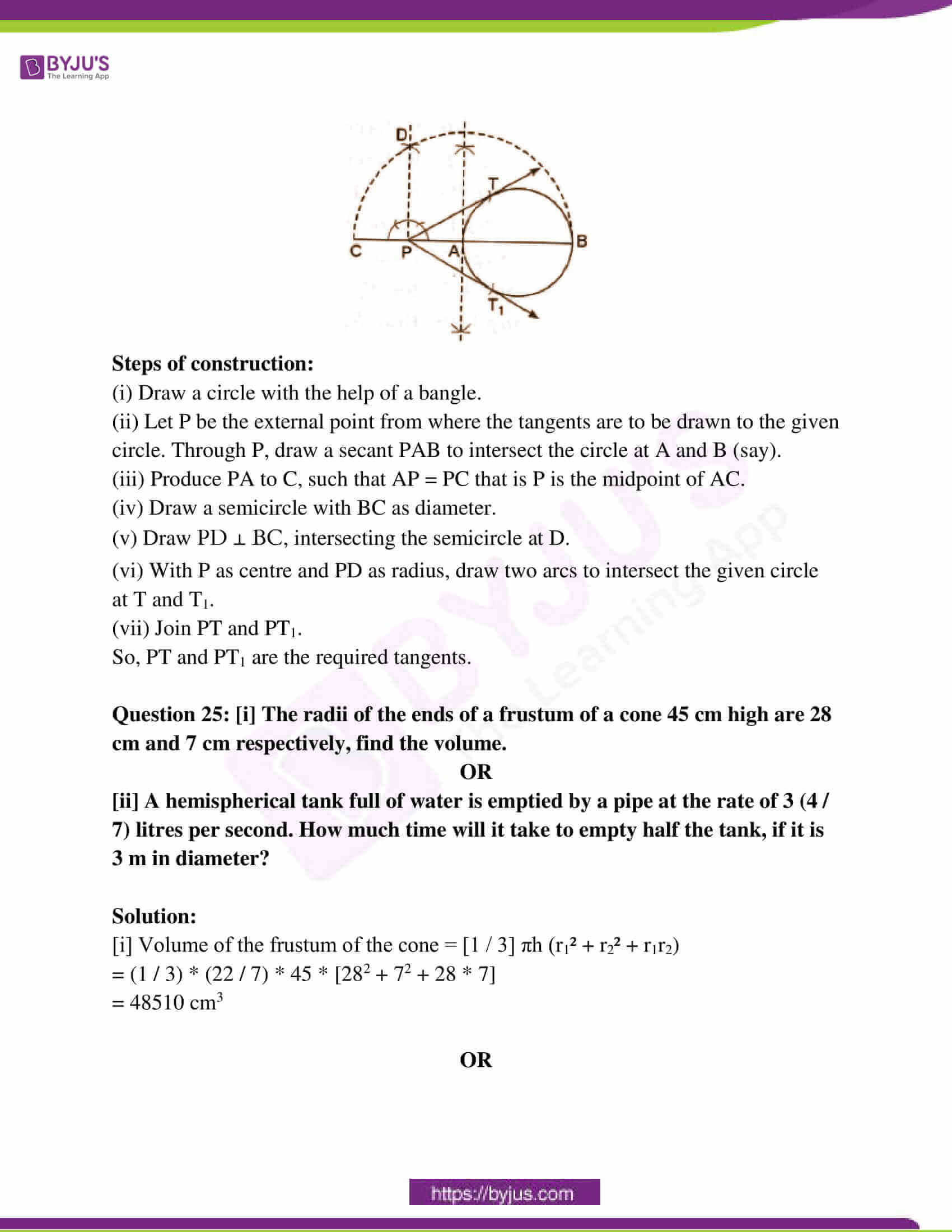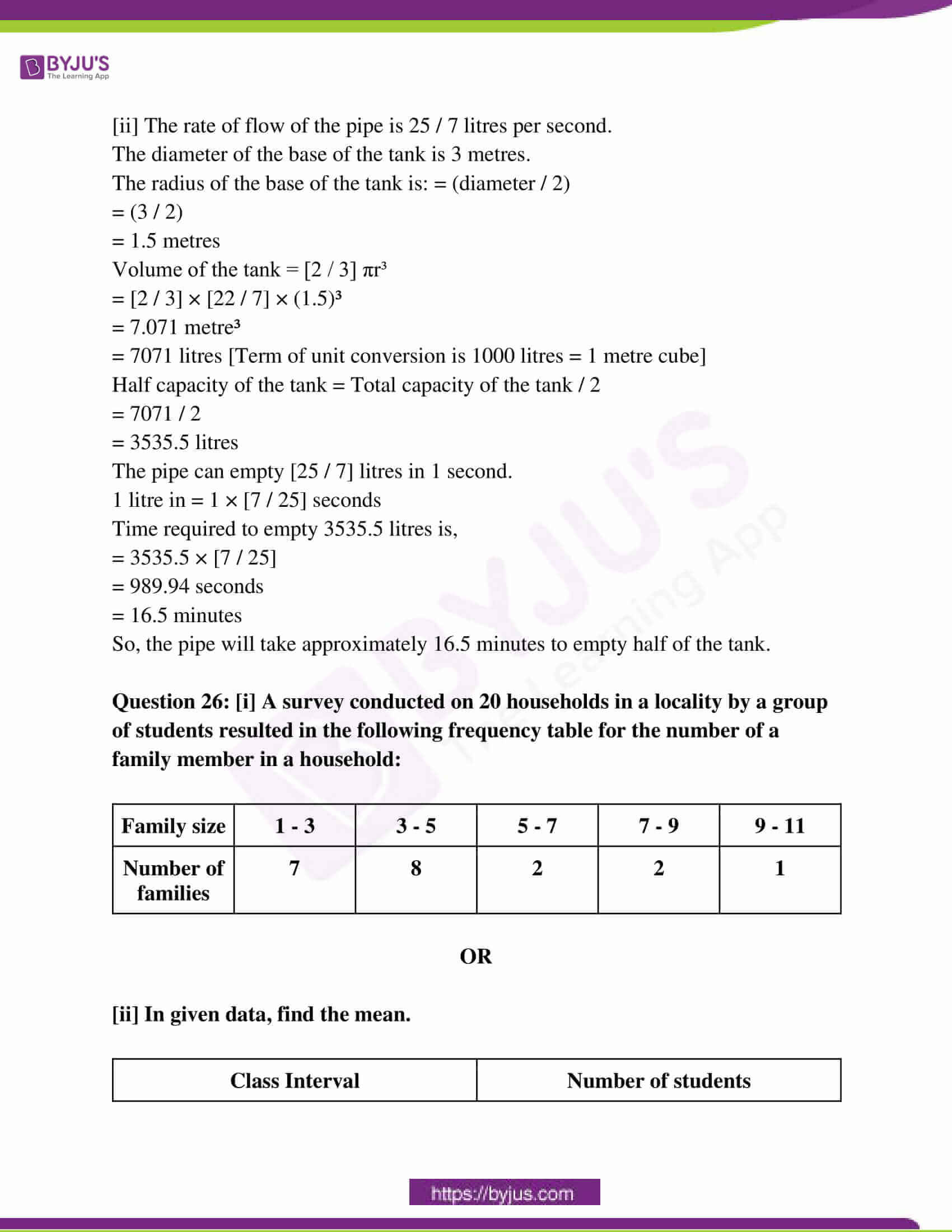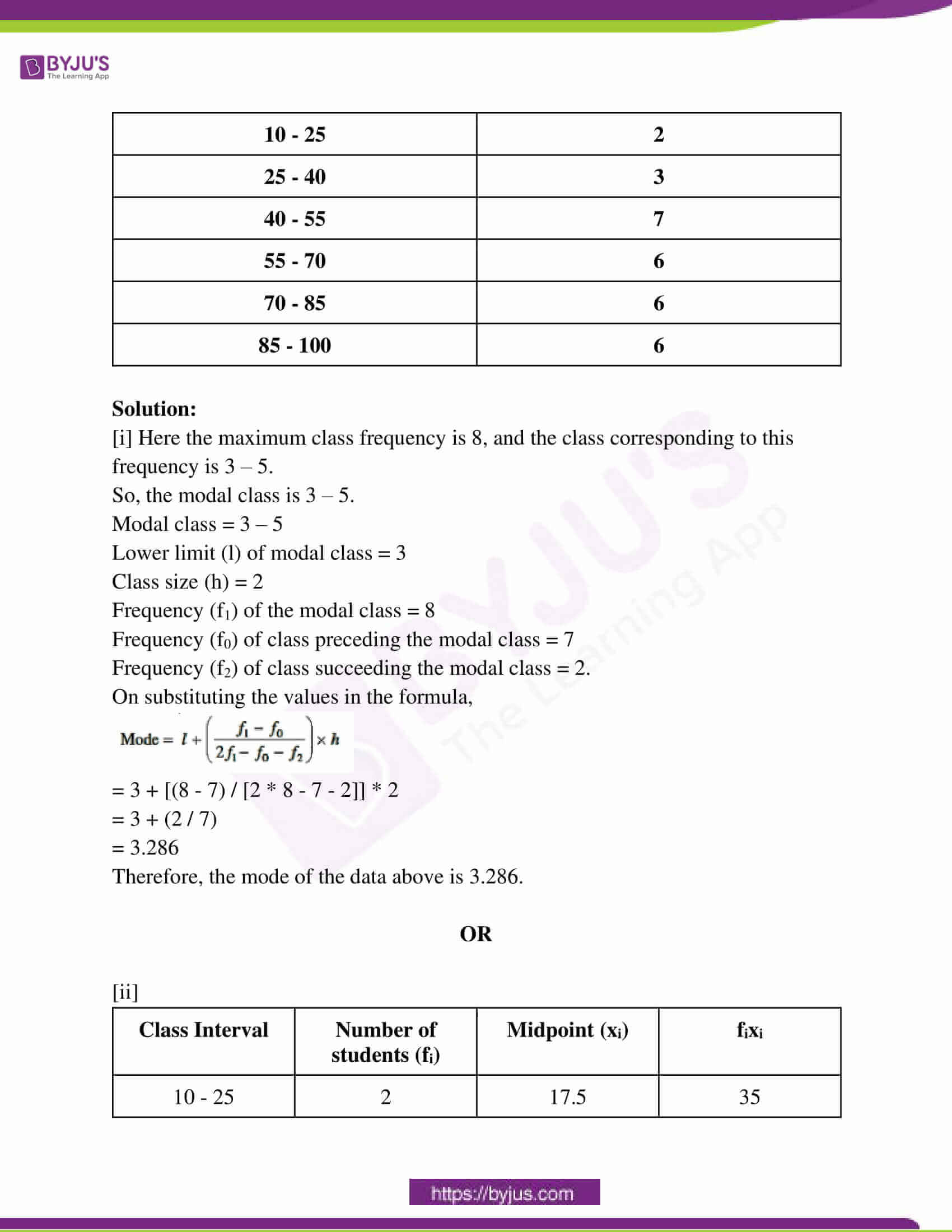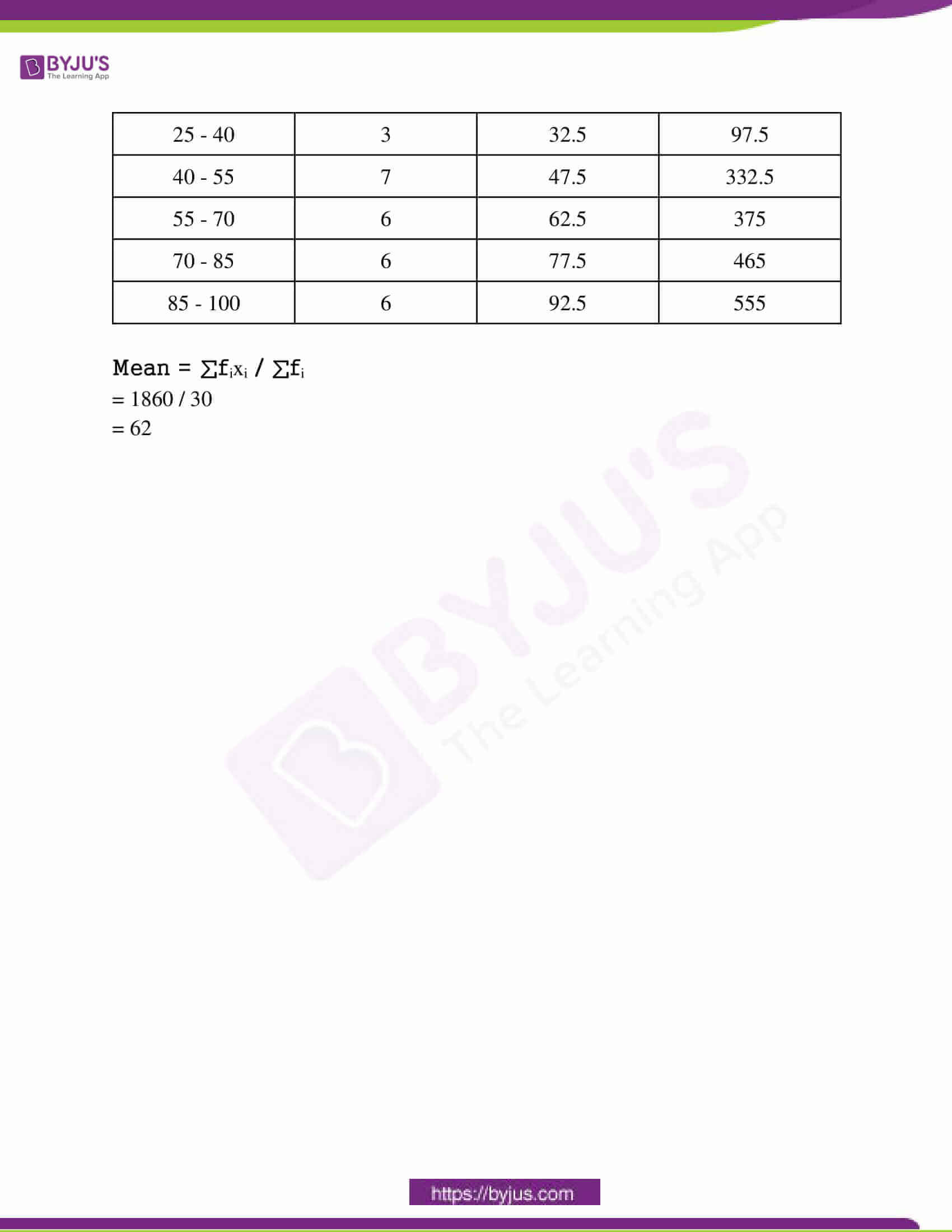(1 * 5 = 5)

Question 1: Choose the correct option and write it in your answer book.

Question i: The H.C.F. of 96 and 404 is :

(a) 120 (b) 4 (c) 10 (d) 3

Question ii: If ɑ and β are the zeroes of the quadratic polynomial ax2 + bx + c, then the value of ɑβ is –

(a) c / a (b) a / c (c) – c / a (d) – a / c

Question iii: The zeroes of the polynomial x2 – 3 will be –

(a) ± √3 (b) ± 3 (c) 3 (d) 9

Question iv: When a1 / a2 = b1 / b2 ≠ c1 / c2 then the system of equation a1x + b1y + c1 = 0 and a2x + b2y + c2 = 0

(a) has two solutions (b) has no solution

(c) has infinitely many solutions (d) has unique solution

Question v: Lines x – 2y = 0 and 3x + 4y – 20 = 0 are:

(a) Intersect (b) Coincide (c) Parallel (d) None

Question 2: Fill in the blanks. (1 * 5 = 5)

[i] A quadratic equation ax2 + bx + c = 0 has no real root if – _______ (D < 0)

[ii] The discriminant of the equation 3x2 – 2x + [1 / 3] = 0 is ______ (D = 0).

[iii] In the AP, 3 / 2, 1 / 2, -1 / 2, 3 / 2 ………… the common difference d is ____. (-1)

[iv] The sum of the probabilities of all the elementary events of an experiment is. _____. (1)

[v] Formula of area of the sector of angle is _____. (𝜋𝑟2θ / 3600)

Question 3: Write true / false in the following. (1 * 5 = 5)

[i] The perpendicular is drawn from the centre of a circle to a chord bisects the chord. [True] [ii] All squares are similar. [True] [iii] Area of right triangle = [1 / 2] × base × altitude. [True] [iv] A line intersecting a circle in two points is called a secant. [True] [v] The angle of elevation of an object viewed is the angle formed by the line of sight with the horizontal when we lower our head to look at the object. [False]

Question 4: Write the answers in one word / sentence. (1 * 5 = 5)

[i] What will be the Arithmetic mean of 1, 2, 3, 4, 5?

Solution: Mean = Sum of the observations / Number of observations

= [1 + 2 + 3 + 4 + 5] / 5

= 15 / 5

= 3

[ii] Write the formula of the median.

Solution:

Median =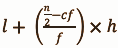[iii] Find the value of Probability of an EVENT E + Probability of the EVENT “not E”.

Solution:

Probability of an “event E” + probability of the event “not E” is P (E) + P (E’).

The sum of the probabilities of an event and its complement is always equal to 1. Probability of an “event E” + probability of the event “not E” = 1

P (E) + P (E’) = 1

[iv] Write the formula of volume of a frustum of a cone.

Solution:

Volume of a conical frustum = V = (1 / 3) * π * h * (r12 + r22 + (r1 * r2)).

[v] How many parallel tangents of a circle?

Solution:

A circle can have exactly two parallel tangents and they must pass through ends of a diameter. That is their point of contacts must be diametrically opposite.

Question 5: Match the following.

Column A Column B

[i] 1 + cot2 θ [a] sin θ

[ii] sec θ [b] 0

[iii] sin2 θ + cos2 θ [c] √3

[iv] tan 60o [d] 1

[v] cos (90 – θ) [e] cosec2 θ

[f] 1 / cos θ

[g] 1 / √3

[i] – e

[ii] – f

[iii] – d

[iv] – c

[v] – a

Question 6: [i] Find the LCM and HCF of 6 and 20 by the prime factorisation method.

OR

[ii] Find the H.C.F. of 6, 72 and 120 using the prime factorisation method.

Solution:

[i] 6 = 2 × 3

20 = 2 × 2 × 5

HCF = 2

LCM = 2 × 2 × 3 × 5 = 60

[ii] 6 = 2 × 3

72 = 2 × 2 × 2 × 3 × 3

120 = 2 × 2 × 2 × 3 × 5

HCF = 2 × 3 = 6

LCM = 2 × 2 × 2 × 3 × 3 × 5 = 360

HCF = 6 and LCM = 360

Question 7: [i] Find a quadratic polynomial, the sum and product of whose zeroes are – 3 and 2 respectively.

OR

[ii] Divide 2x2 + 3x + 1 by x + 2.

Solution:

[i] Let α and β be the two roots of the equation

α + β = -3

α β = 2

A quadratic polynomial can be written in the form:

P (x) = x2 – (sum of roots) x + product of roots

P (x) = x2 – (α + β) x + α β = 0

P (x) = x2 – (-3) x + 2 = 0

x2 + 3x + 2 = 0 is the polynomial.

OR

[ii]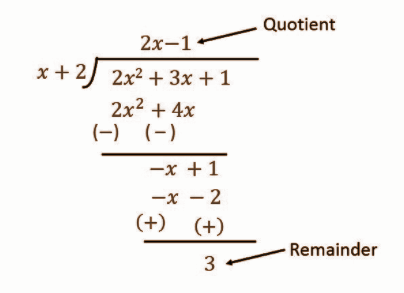Question 8: [i] Find the distance between points (2, 3) and (4, 1).

OR

[ii] Find the area of a triangle whose vertices (1, – 1), (– 4, 6) and – 3, – 5).

Solution:

[i] The distance between two points (2, 3) & (4, 1) is given by

D = √(x2 – x1) + (y2 – y1)2

= √(4 – 2)2 + (1 – 3)2

= √22 + 22

= √8

= 2√2

OR

[ii] Side 1² = (-4-1)² + (6-(-1))² = (-5)² + 7² = 25 + 49 = 74

Side 2² = (-3-1)² + (-5 -(-1))² = (-4)² + (-4)² = 16 + 16 = 32

Side 3² = (-3 -(-4))² + (-5 – 6)² = 1² + (-11)² = 1 + 121 = 122

Side 1 = √74 = 8.60

Side 2 = √32 = 5.66

Side 3 = √122 = 11.05

S = (Side 1 + Side 2 + Side 3) / 2

= 25.31/2

= 12.66

Area = √(s (s – side 1)(s – side 2)(s – side 3)

= √(12.66 * 4.06 * 7 * 1.61)

= √579.27

= 24

Question 9: [i] Two players Sangeeta and Reshma, play a tennis match. It is known that the probability of Sangeeta winning the match is 0.62. What is the probability of Reshma winning the match?

OR

[ii] A box contains 3 blue, 2 white and 4 red marbles. If a marble is drawn at random from the box, what is the probability that will be

(i) white?

(ii) blue?

(iii) red?

Solution:

[i] The probability of Sangeeta winning the match is 0.62 and the probability of Reshma winning the match be P (not E) i.e it will be the probability of Sangeeta not winning the match.

P (E) + P (not E) = 1

=> 0.62 + P (not E) = 1

=> P (not E) = 0.38

Hence, the probability of Reshma winning the same match is 0.38.

OR

[ii] Total number of marble = 3 + 2 + 4 = 9

(i) The probability of getting a white ball = 2 / 9

(ii) The probability of getting a blue ball = 3 / 9 = 1 / 3

(iii) The probability of getting a red ball = 4 / 9.

Question 10: [i] If P(E) = 0.05, what is the probability of (“not E”)?

OR

[ii] One card is drawn from a well-shuffled deck of 52 cards. Calculate the probability, that the card will –

(i) be an ace

(ii) not be an ace.

Solution:

[i] P (not E) = 1 – P (E)

= 1 – 0.05

= 0.95

[ii] (i) P (getting an ace card) = Number of aces / Total number of cards

= 4 / 52

= 1 / 13

(ii) P (not getting an ace card) = 1 – P (getting an ace card)

= 1 – (1 / 13)

= 12 / 13

Question 11: [i] Prove that √1 + sin A / 1 – sin A = sec A + tan A

OR

[ii] Evaluate the following : sin 60°. cos 30° + sin 30°. cos 60°.

Solution:

[i] LHS = √(1 + sinA) / √(1 – sinA)

= √(1 + sinA) × √(1 + sinA)/√(1 – sinA) × √(1 + sinA)

= √(1 + sinA)² / √(1 – sin²A)

= (1 + sinA) / √cos²A

= (1 + sinA) / cosA

= 1 / cosA + sinA / cosA

= secA + tanA

= RHS

OR

[ii] sin 60°. cos 30° + sin 30°. cos 60°

= sin 60 * cos (90 – 60) + sin 30 . cos (90 – 30)

= sin 60 * sin 60 + sin 30 * sin 30

= sin² 60 + sin² 30

= {√3 / 2}² + [1 / 4]

= [3 / 4] + [1 / 4]

= (3 + 1) / 4

= 4 / 4

= 1

Question 12: [i] Find the value of K, if the points A (2, 3), B (4, K) and C (6, – 3) are collinear.

OR

[ii] Find the ratio in which the y-axis divides the line segment joining the points (5, – 6) and (– 1, – 4) also find the point of intersection.

Solution:

[i] The given points are A (2, 3), B (4, k ) and C (6, -3).

Here, (x1 = 2 , y1 = 3) , (x2 = 4, y2 = k) and (x3 = 6, y3 = -3).

It is given that the points A, B and C are collinear.

Then,

x1 (y2 – y3) + x2 (y3 – y1) + x3 (y1 – y2) = 0

2 (k + 3) + 4 (- 3 – 3) + 6 (3 – k) = 0

2k + 6 – 24 +18 – 6k = 0

– 4k = 0

k = 0

OR

[ii] Let the coordinates of the point be (0, y) and the y-axis divides the line segment in the ratio m:n.

So,

x = [mx2 + nx1] / [m + n]

x = 0 (given)

0 = m * 5 + n * -1 / [m + n]

0 = 5m – n

5m = n

m / n = 1 / 5

m : n = 1 : 5

y = [my2 + my1] / [m + n]

y = 1 * -6 + 5 * – 4 / 1 + 5

y = – 6 – 20 / 6

y = – 26 / 6

y = – 13 / 3

The coordinates of the point are (0, – 13 / 3).

Question 13: [i] The length of tangents drawn from an external point to a circle is equal.

OR

[ii] Prove that the tangents drawn at the ends of a diameter of a circle are parallel.

Solution:

[i]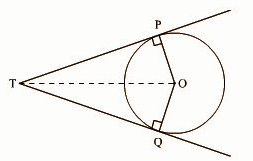Let the two tangents be PT and QT. They are drawn to the circle of centre O as shown in the figure.

The tangents PT and QT touch to the circle at P and Q, respectively.

To prove: Length of PT = Length of QT

Construction:- Draw a line segment OT, from centre O to external point T {touching point of two tangents}.

Now ∆POT and ∆QOT are obtained.

The tangents make right angles with the radius of the circle.

So, ∠OPT = ∠OQT = 90°

Now, it is clear that both the triangles ∆POT and QOT are right-angled triangles and there exists a common hypotenuse OT.

In ∆POT and ∆QOT,

∠OPT = OQT = 90°

Common hypotenuse OT and OP = OQ [OP and OQ are radii]

So, by RHS rule of similarity,

∆POT ~ ∆QOT

Hence, OP / OQ = PT / QT = OT / OT

PT / QT = 1

PT = QT

OR

[ii]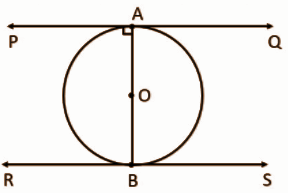Given: A circle with centre O and diameter AB.

Let PQ be the tangent at point A & RS be the tangent at point B.

To Prove: PQ || RS

Since PQ is tangent at point A, OA⟂PQ (Tangent at any point of a circle is perpendicular to the radius through the point of contact)

∠OAP = 90° … (i)

Similarly

RS is a tangent at point B, OB⟂RS (Tangent at any point of a circle is perpendicular to the radius through the point of contact)

∠OBS = 90° … (ii)

From (i) and (ii)

∠OAP = 90° and ∠OBS = 90°

∠OAP =∠OBS

∠BAP = ∠ABS

For lines PQ and RS and transversal AB, ∠BAP=∠ABS i.e., both alternate angles are equal.

So, lines are parallel

∴ PQ || RS

Question 14: [i] Find the area of the sector of a circle with radius 4 cm and angle 30°. Also, find the area of the corresponding major sector. (Use = 3.14)

OR

[ii] Find the area of a sector of a circle with radius 6 cm whose angle of the sector is 60°.

Solution:

[i] Area of Sector = [θ / 360] × ∏ × r²

θ = 30

r = 4 cm

Area of Sector……..

= [30 / 360] × [22 / 7] × (4)²

= [1 / 12] × [22 / 7] × 16

= 352 / 84

= 4.19 cm ²

Area of the major corresponding sector = Area of Circle – Area of Sector

= ∏r² – 4.19 cm²

= (22 / 7 × 16) – 4.19 cm²

= 50.29 – 4.19 cm²

= 46.09 cm²

OR

[ii] Area of sector = [Φ / 360°] * πr²

Φ = angle of sector = 60o

Area = 6 * 6 * [22 / 7] * 6

= 792 / 42

= 18.85

Area of sector = 18.85cm²

Question 15: [i] Prove that 5 – √3 is an irrational number.

OR

[ii] Show that any positive odd integer is of the form 4q + 1 or 4q + 3 where q is an integer.

Solution:

[i] Let us assume the given number be rational and we will write the given number in p/q form

⇒ 5 − √3 = p / q

⇒ √3 = [5q − p] / q

LHS is irrational and RHS is rational, which is not possible.

Hence our assumption that the given number is rational is false.

⇒ 5 − √3 is irrational.

OR

[ii] Let a be any positive integer and b = 4. Then by Euclid’s algorithm,

a = 4q + r for some integer q ≥ 0, and r = 0, 1, 2, 3

Case 1: When r = 0,

a = 4q

Case 2: When r = 1,

a = 4q + 1

Case 3: When r = 2,

a = 4q + 2

When r = 3,

a = 4q + 3

Since, 4q and 4q + 2 are multiples of 2, they are even numbers.

Now, 4q that is 2(2q) is an even number.

∴4q + 1 is an odd number.

4q + 2 that is 2(2q + 1) which is also an even number.

∴ (4q + 2) + 1 = 4q + 3 is an odd number.

Thus, it is proved that any odd integer can be written in the form 4q + 1 or 4q + 3 where q is some integer.

Question 16: [i] Find the zeros of the quadratic polynomial x2 + 7x + 10 and verify the relationship between the zeros and the coefficients.

OR

[ii] Divide 3x3 + x2 + 2x + 5 by 1 + 2x + x2 .

Solution:

[i] Let p(x) = x² + 7x + 10

To zeroes of the polynomial, p(x) = 0

=> x² + 7x + 10 = 0

=> x² + 2x + 5x + 10 = 0

=> x( x + 2 ) + 5( x + 2 ) = 0

=> ( x + 2 )( x + 5 ) = 0

=> x + 2 = 0 or x + 5 = 0

=> x = -2 or x = -5

The zeroes of p(x) are -2, -5.

i) Compare x² + 7x + 10 with ax² + bx + c,

a = 1 , b = 7 , c = 10

Sum of the zeroes =

– 2 – 5 = ( -b / a )

-7 = -7 / 1

-7 = -7

ii) Product of the zeroes

-2 * -5 = c / a

10 = (10) / (1)

10 = 10

OR

[ii]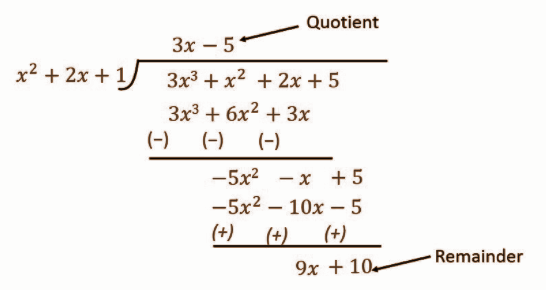Question 17: [i] If the sum of the first 14 terms of an A.P. is 1050 and its first term is 10, find the 20th term.

OR

[ii] Find the 31st term of an A.P. whose 11th term is 38 and 16th term is 73.

Solution:

[i] S14 = 1050

n = 14, a = 10

Sn = [n / 2] [2a + (n – 1)d]

1050 = [14 / 2] [20 + 13d]

1050 = 140 + 91d

910 = 91d

d = 10

Therefore,

a20 = 10 + (20 – 1) × 10

= 200

The 20th term is 200.

OR

[ii] a11 = 38 and a16 = 73

an = a + (n – 1)d

a11 = a + 10d = 38 and a16 = a + 15d = 73

Subtracting 11th term from 16th term,

a + 15d – a – 10d = 73 – 38

5d = 35

d = 7

Substituting the value of d in 11th term,

a + 10 x 7 = 38

a + 70 = 38

a = 38 – 70

= – 32

a31 = a + 30d

= – 32 + 30 x 7

= – 32 + 210

= 178

Question 18: [i] From a point P on the ground the angle of elevation of the top of a 10-meter tall building is 30°. A flag is hoisted at the top of the building and the angle of elevation of the top of the flagstaff from P is 45o. Find the length of the flagstaff and the distance of the building from point P.

OR

[ii] The angle of depression of the top and the bottom of an 8 m. tall building from the top of a multi-storeyed building is 30°and 45° respectively. Find the height of the multi-storeyed building and distance between the two buildings.

Solution:

[i]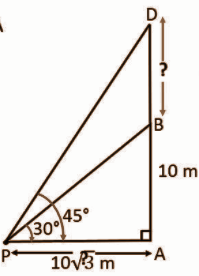In ΔBAP,

tan 30° = BA / PA

1 / √3 = 10 / PA

1 * PA = 10 * √3

PA = 10√3 m

PA = 10 * 1.732

= 17.32m

IN ΔDPA,

tan 45° = AD / AP

1 = DB + BA / 10√3

==> 10√3 = 10 + BD

==> BD = 10√3 – 10

==> BD = 10 [√3 – 1]

BD = 10 * (1.732 – 1)

= 7.32m

OR

[ii]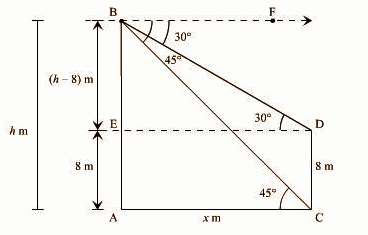Let AB and CD be the multi-storeyed building and the building respectively.

Let the height of the multi-storeyed building = ‘h’ m and the distance between the two buildings = ‘x’ m.

AE = CD = 8 m

BE = AB – AE = (h – 8) m and AC = DE = x m [Given]

∠FBD = ∠BDE = 30° (Corresponding angles)

∠FBC = ∠BCA = 45° (Corresponding angles)

In Δ ACB,

tan 45o = AB / AC

1 = h / x

x = h —- (i)

In Δ BDE,

tan 30o = BE / ED

1 / √3 = [h – 8] / x

x = √3 [h – 8] —– (ii)

From (i ) and (ii),

h = √3h – 8√3

√3h – h = 8√3

h = 8√3 / √3 – 1

h = [8√3 / √3 – 1] * [√3 + 1 / √3 + 1]

= 4√3 (√3 + 1)

= 12 + 4√3 m

Distance between the two-building, x = 12 + 4√3 m.

Question 19: [i] Use the elimination method to find all possible solutions of the following pair of linear equations :

2x + 3y = 8

4x + 6y = 7

OR

[ii] The cost of 5 oranges and 3 apples is Rs. 35 and the cost of 2 oranges and 4 apples are Rs. 28. Let us find the cost of an orange and an apple.

Solution:

[i] 2x + 3y = 8 ……(1)

4x + 6y = 7 …..(2)

Step 1: Multiply equation (1) by 2 and equation (2) by 1 to make the coefficients of x equal.

Then we get the equations as :

4x + 6y = 16 ……(3)

4x + 6y = 7 ………(4)

Step 2 : Subtracting equation (4) from equation (3),

(4x – 4x) + (6y – 6y) = 16 – 7

0 = 9, which is a false statement.

Therefore, the pair of equations has no solution.

OR

[ii] Let the cost of 1 orange be x and the cost of 1 apple be y.

According to question

5x + 3y = 35 – – – – – (i)

2x + 4y = 28 – – – – (ii)

Multiply by 2 in equation (i) and by 5 in equation (ii),

=> 10x + 6y = 70 – – – – (i)

=> 10x + 20y = 140 – – – (ii)

By subtraction equation (i) from (ii)

=> 14y =70

=> y = 70 / 14

=> y = 5

Putting the value of y in equation (i)

=> 5x + (3 * 5) = 35

=> 5x = 35 – 15

=> x = 20 / 5

=> x = 4

Hence

The cost of an orange = ₹4 and the cost of an apple = ₹5.

Question 20: [i] Observe the figure and find ∠P.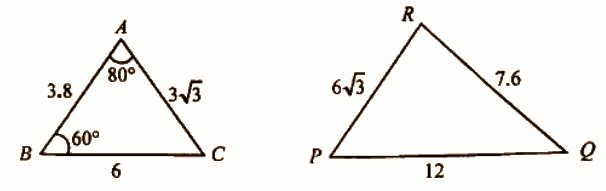OR

[ii] ABC is an Isosceles triangle right angled at C. Prove that AB2 = 2 AC2.

Solution:

[i] In ΔABC and ΔPQR,

AB / QR = AC / RP = BC / PQ = 1/2 (each).

If in two triangles, the ratio of sides is equal then triangles are similar.

ΔABC ~ ΔRQP

∠A = ∠R = 80°

∠B = ∠Q = 60°

∠C = ∠P = 180° – (80° + 60°)

= 180° – 140°

= 40°

OR

[ii] Given: ΔABC is a right triangle.

Also, ΔABC is an isosceles triangle.

To prove: AB² = 2AC²

Here,

Hypotenuse = AB

Also, as it is given that, ΔABC is isosceles, AC = BC [equal sides of isosceles Δ]

Using Pythagoras theorem,

In Δ ABC,

AB² = AC² + BC²

AB² = AC² + AC² [AC = BC]

AB² = 2 AC²

Question 21: [i] Find the area of the shaded region in the given figure where ABCD is a square of side 14 cm.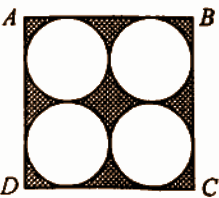OR

[ii] In a circle of radius 21 cm, an arc subtends an angle of 60° at the centre find;

(i) the length of the arc

(ii) area of the sector.

Solution:

[i] Area of square ABCD = a2

= 14 × 14

= 196 cm²

Diameter of each circle = radius / 2

= 14 / 2

= 7 cm

So, the radius of each circle = 7 / 2 cm

So, area of 4 circles = 4𝛑r2

= 4 × [22 / 7] × [7 / 2] × [7 / 2]

= 4 × [154 / 4] cm²

= 154 cm²

Area of shaded region = Area of a square – Area of 4 circles

= 196 – 154

= 42 cm²

Hence, the area of the shaded region is 42 cm².

OR

[ii] Radius (r) of circle = 21 cm

The angle subtended by the given arc = 60°

Length of an arc of a sector of angle = (2𝜋𝑟θ / 3600)

Length of arc ACB = [60o / 360o] * 2 * (22 / 7) * (21)

= [1 / 6] * 2 * 22 * 3

= 22 cm

Area of sector OACB = (𝜋𝑟2θ / 3600)

= [60o / 360o] * (22 / 7) * (21)2

= [1 / 2] * 22 * 3 * 7

= 11 * 3 * 7

= 231 cm2

Question 22: [i] Find the roots of the following equation: x + [1 / x] = 3, x ≠ 0.

OR

[ii] Find two consecutive odd positive integers, the sum of whose squares is 290.

Solution:

[i] x + [1 / x] = 3

x2 + 1 = 3x

x2 – 3x + 1 = 0

Here a = 1, b = -3, c = 1

x = -b ± √b2 – 4ac / [2a]

= -(-3) ± √9 – 4 * 1 * 1 / [2 * 1]

= 3 ± √5 / 2

x = 3 + √5 / 2 and x = 3 – √5 / 2

OR

[ii] Let the two consecutive odd positive integers be x and x + 2

The sum of the squares is 290.

⇒ x² + ( x + 2 )² = 290

⇒ x² + x² + 4x + 4 = 290

⇒ 2x² + 4x + 4 – 290 = 0

⇒ 2x² + 4x – 286 = 0

Dividing by 2,

⇒ x² + 2x – 143 = 0

⇒ x² + 13x – 11x – 143 = 0

⇒ x ( x + 13 ) -11 ( x + 13 ) = 0

⇒ ( x – 11 ) ( x + 13 ) = 0

⇒ x = -13, 11

In the question, we are given with positive integers.

Hence, the number is 11 and the consecutive odd number is 13.

Question 23: [i] If sin A = 3 / 4, calculate cos A and tan A.

OR

[ii] Evaluate the following: 2 tan2 – 45° + cos2 30° – sin2 60°.

Solution:

[i] The three fundamental trigonometric ratios are stated as:

sin θ = Opposite side / Hypotenuse side

tan θ = Opposite side / Adjacent side

cos θ = Adjacent side / Hypotenuse side

sin A = 3 / 4

To calculate the length of the third side of the triangle, use the Pythagorean theorem to determine the two remaining trigonometric ratios.

The length of the third side will be:

a = √42 − 32

a = √7

Therefore:

tan A = 3 / √7

cos A = √7 / 4

OR

[ii] 2 tan² 45° + cos² 30° – sin² 60°

tan 45° = 1

cos 30° = (√3 / 2)

sin 60° = (√3 / 2)

So put the values in the equation,

2 tan² 45° + cos² 30° – sin² 60°

= 2 (1)² + (√3 / 2)² – (√3 / 2)²

= 2 × 1 + (3 / 4) – (3 / 4)

= 2 + (3 – 3) / 4

= 2 + (0 / 4)

= 2 + 0

= 2

2 tan² 45° + cos² 30° – sin² 60° = 2

Question 24: [i] Construct a triangle similar to a given triangle ABC with its side equal to 5 / 3 of the corresponding sides of the triangle ABC.

OR

[ii] Draw a circle with the help of a bangle. Take a point outside the circle. Construct the pair of tangents from this point to the circle.

Solution:

[i]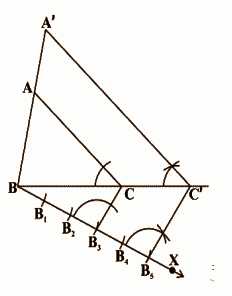Steps of construction:

(i) Draw any ray BX making an acute angle with BC on the side opposite to the vertex A.

(ii) From B cut off 5 arcs B1, B2, B3, B4, B5 on BX such that BB1 = B1B2 = B2B3 = B3B4 = B4B5.

(iii) Join B3 to C and draw a line through B5 parallel to B3C, intersecting the extended line segment BC at C’.

(iv) Draw a line through C’ parallel to CA intersecting the extended line segment BA at A’.

Then A’B’C’ is the required triangle.

OR

[ii]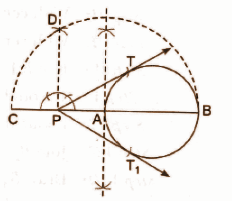Steps of construction:

(i) Draw a circle with the help of a bangle.

(ii) Let P be the external point from where the tangents are to be drawn to the given circle. Through P, draw a secant PAB to intersect the circle at A and B (say).

(iii) Produce PA to C, such that AP = PC that is P is the midpoint of AC.

(iv) Draw a semicircle with BC as diameter.

(v) Draw PD ⟂ BC, intersecting the semicircle at D.

(vi) With P as centre and PD as radius, draw two arcs to intersect the given circle at T and T1.

(vii) Join PT and PT1.

So, PT and PT1 are the required tangents.

Question 25: [i] The radii of the ends of a frustum of a cone 45 cm high are 28 cm and 7 cm respectively, find the volume.

OR

[ii] A hemispherical tank full of water is emptied by a pipe at the rate of 3 (4 / 7) litres per second. How much time will it take to empty half the tank, if it is 3 m in diameter?

Solution:

[i] Volume of the frustum of the cone = [1 / 3] πh (r1² + r2² + r1r2)

= (1 / 3) * (22 / 7) * 45 * [282 + 72 + 28 * 7]

= 48510 cm3

OR

[ii] The rate of flow of the pipe is 25 / 7 litres per second.

The diameter of the base of the tank is 3 metres.

The radius of the base of the tank is: = (diameter / 2)

= (3 / 2)

= 1.5 metres

Volume of the tank = [2 / 3] πr³

= [2 / 3] × [22 / 7] × (1.5)³

= 7.071 metre³

= 7071 litres [Term of unit conversion is 1000 litres = 1 metre cube]

Half capacity of the tank = Total capacity of the tank / 2

= 7071 / 2

= 3535.5 litres

The pipe can empty [25 / 7] litres in 1 second.

1 litre in = 1 × [7 / 25] seconds

Time required to empty 3535.5 litres is,

= 3535.5 × [7 / 25]

= 989.94 seconds

= 16.5 minutes

So, the pipe will take approximately 16.5 minutes to empty half of the tank.

Question 26: [i] A survey conducted on 20 households in a locality by a group of students resulted in the following frequency table for the number of a family member in a household:

 Family size 1 – 3 3 – 5 5 – 7 7 – 9 9 – 11 Number of families 7 8 2 2 1

OR

[ii] In given data, find the mean.

 Class Interval Number of students 10 – 25 2 25 – 40 3 40 – 55 7 55 – 70 6 70 – 85 6 85 – 100 6

Solution:

[i] Here the maximum class frequency is 8, and the class corresponding to this frequency is 3 – 5.

So, the modal class is 3 – 5.

Modal class = 3 – 5

Lower limit (l) of modal class = 3

Class size (h) = 2

Frequency (f1) of the modal class = 8

Frequency (f0) of class preceding the modal class = 7

Frequency (f2) of class succeeding the modal class = 2.

On substituting the values in the formula,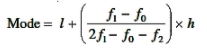= 3 + [(8 – 7) / [2 * 8 – 7 – 2]] * 2

= 3 + (2 / 7)

= 3.286

Therefore, the mode of the data above is 3.286.

OR

[ii]
 Class Interval Number of students (fi) Midpoint (xi) fixi 10 – 25 2 17.5 35 25 – 40 3 32.5 97.5 40 – 55 7 47.5 332.5 55 – 70 6 62.5 375 70 – 85 6 77.5 465 85 – 100 6 92.5 555

Mean = ∑fixi / ∑fi

= 1860 / 30

= 62